# Incorporating treatment non-compliance into call-ins

I have previously published work on identifying optimal individuals to prioritize for call-ins in Focused Deterrence interventions. The idea is we want to identify optimal people to spread the message, so you call in a small number of individuals and they should spread the message to the remaining group. There are better people than others to seed the message to to make sure it spreads throughout the network.

I knew of a direct improvement on that algorithm I published (very similar to the TURF problem I described the other day). But the bigger issue was that even when you call in individuals they do not always come to the meeting – treatment non-compliance. When working with state parole and/or local probation, the police department can ask those agencies to essentially make people come in, but otherwise it is voluntary.

The TURF problem I did the other day gave me a bit of inspiration on how to tackle that treatment non-compliance problem though. In a nutshell when you calculate whether someone is reached (via being directly connected to someone called-in), they can be partially reached based on the probability of the selected nodes treatment compliance. I have posted the code to follow along on dropbox here. I won’t go through the whole thing, but just some highlights.

# The Model

First, in some quick and dirty text math, the model is:

`Maximize Sum( R_i )`

Subject to:

• `R_i <= Sum( S_j*p_j ) for each i`
• `Sum( S_j ) = k`
• `S_i element of [0,1]`
• `R_i <= 1 for each i`

Here `i` refers to an individual node in the gang/group network.

The first constraint `R_i <= Sum( S_j*p_j )`, the j’s are the nodes that are connected to i (and i itself). The p_j are the estimates that an individual will comply with coming into the call-in. For one agency we worked with for that project, they guessed that those who don’t need to come in comply about 1/6th of the time, so I use that estimate here in my examples, and give people who are on probation/parole a 1 for the probability of compliance.

Second constraint is we can only call in so many people, here `k`. The model solves very fast, so you can generate results for various k until you get the reach you want to in the end. (You could do the model the other way, minimize `S_i` while constraining the minimized acceptable reach, e.g. `Sum( R_i ) >= threshold`, I don’t suggest this in practice though, as when dealing with compliance there may be no feasible solution that gets you the amount of reach in the network you want.)

For the third constraint, the decision variables `S_i` are binary 0/1’s, but the `R_i` are continuous. But the trick here is that the last constraint, `R_i <= 1`, means that the expected reach is capped at 1. Here is a way to think about this, imagine you want to know the chance that person A is reached, and they are connected to two called-in individuals, who each have a 40% chance at complying with the treatment (coming to the call-in). The expected times person A would be reached then is additive in the probabilities, `0.4 + 0.4 = 0.8`. If we had 3 people connected to A again at 40% apiece, the expected number of times A would be reached is then `0.4 + 0.4 + 0.4 = 1.2`. So a person can be reached multiple times. (Note this is not the probability a person is reached at least once! It is a non-linear problem to model that.)

But if we took away the last constraint, what would happen is that the algorithm would just pick the nodes that had the highest number of neighbors. Since we are maximizing expected reach, if we had a sample of two people, the expected reach values of `[2.5, 0]` would be preferable to `[1, 1]`, although clearly we rather have the reach spread out. So to prevent that, I cap the expected reach variable at 1, `R_i <= 1 for each i`, so this spreads out the selected individuals. So in the end the expected number of times people are reached are a lower bound estimate, but those are only people who are expected to receive the message multiple times.

This is a bit of a hack, but in my tests works quite well. I attempted to model the non-linear problem of estimating the probabilities at the person level and still maximizing the expected reach (in the code I have an example of using the `CVXR` R package). But it was quite fickle in when it would return a solution. So I am focusing on the linear program here, which is not perfect, but is an improvement over my prior published work.

# Some Python Snippets

So for my example code, I am using City 4 Gang 4 from my paper. The reason is this was the largest network, and my original algorithm performed the worst. 99 nodes, and my original algorithm identified a 33 person dominant set, but Borgotti’s tool (that uses a genetic algorithm) identified a 29 dominant set.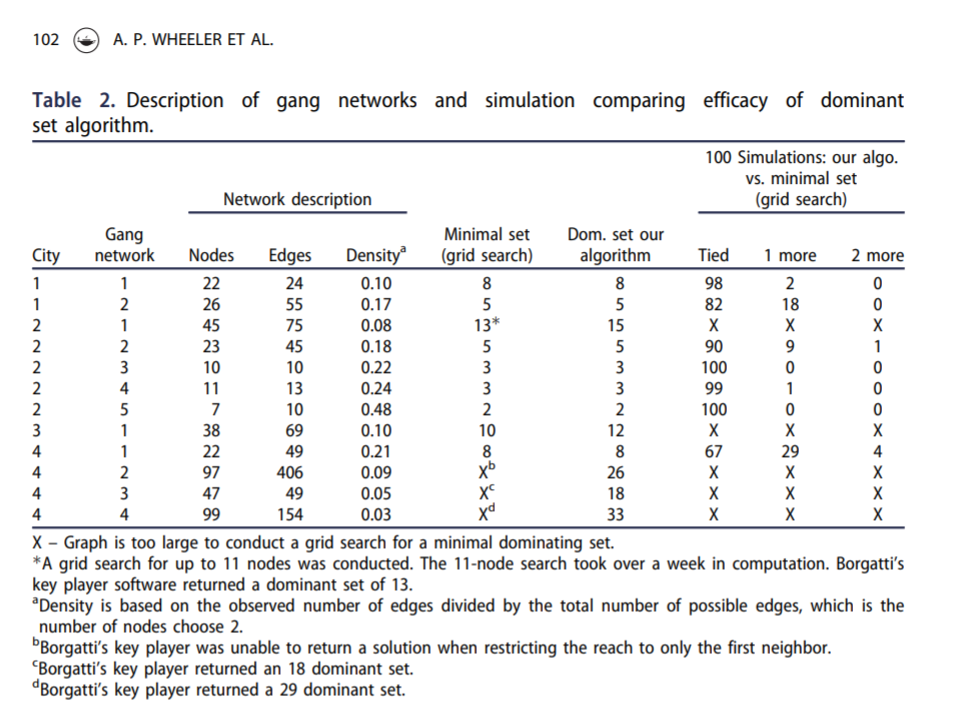Here is an example of calling my function to select the individuals for a call-in based on the non-compliance estimates. (`g4` is the networkx graph object, the second arg is the number of individuals, and `compliance` is the node attribute that has the probability of treated compliance.) If we call in only 5 people, we still expect a reach of 29 individuals. Here there ends up being some highly connected people on parole/probation, so they have a 1 probability of complying with the treatment.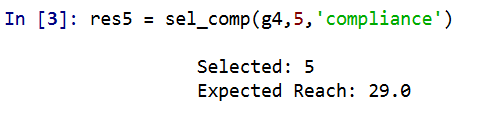A consequence of this algorithm is that if you pipe in 1’s for the treatment compliance, you basically get an improvement to my original algorithm. So for a test we can see if I get the same minimal dominating set as Borgotti did for his algorithm here, where `const` is just everybody complies 100% of the time.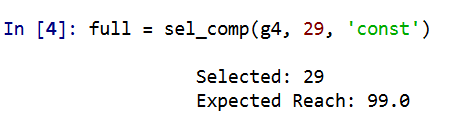And yep we get a dominating set (all 99 people are reached). What happens if we go down one, and only select 28 people?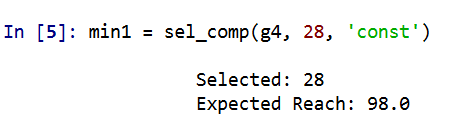We only reach 98 out of the 99. So it appears a 29 set is the minimal dominating set here. But like I said the treatment non-compliance is a big deal in this setting. What is our expected reach if we take that into account, but still call-in 29 people?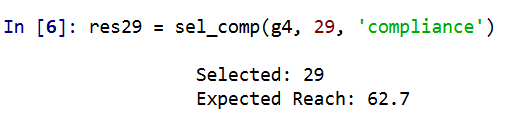It is still pretty high, around 2/3s of the network, but is still much smaller. Also if you look at the overlap between the constant versus non-compliance model, they select quite a few different individuals. It makes a big difference.

Here is a graph I made of selecting 20 individuals. Red means I selected that person, pink means they are reached at least some, and the size of the reach is proportion to the node. Then grey folks I wouldn’t expect to be reached by the message (at least by first degree connections).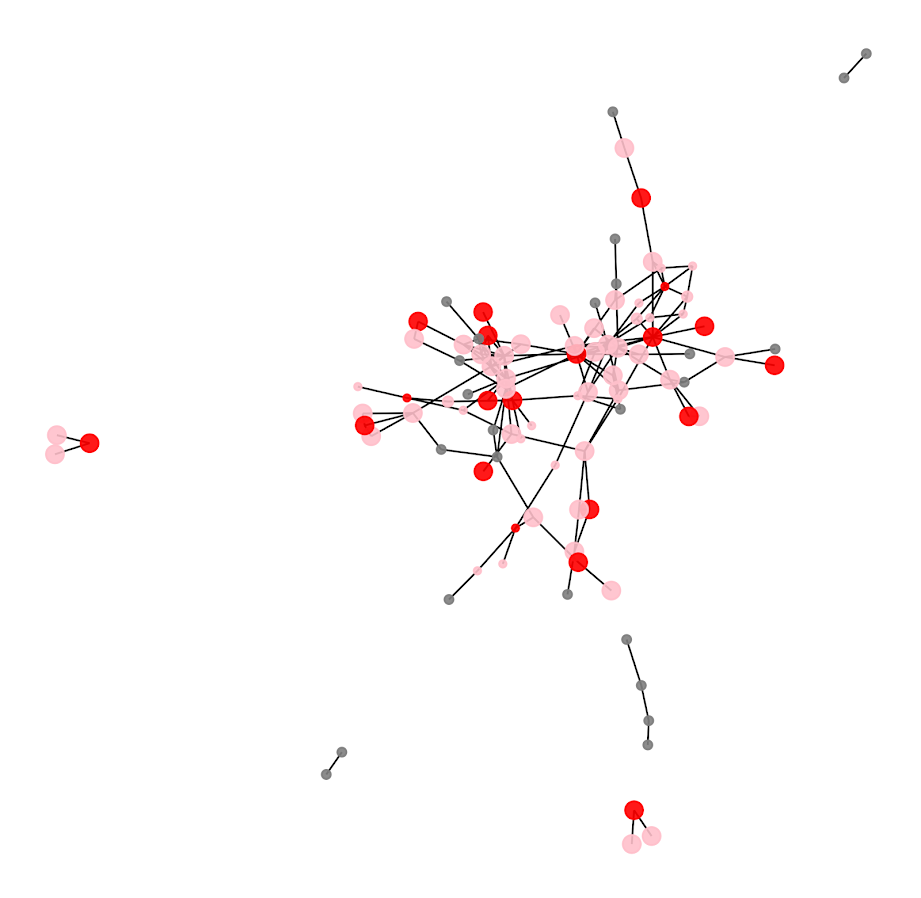So you can see that most of the people selected have that full 1 expected reach, so the algorithm does prioritize individuals on probation/parole who have a 100% expected compliance. But you can see a few folks who have a lower compliance who are selected as they are in places in the network not covered by those on probation/parole.

I have a tough time getting network layouts to look nice in python (even with the same layout algorithms, I feel like igraph in R just looks much better out of the box).

# Future Work

Out of the box, this algorithm could incorporate several different pieces of information. So here I use the non-compliance estimate as a constant, but you could have varying estimates for that based on some other model no problem (e.g. older individuals comply more often than younger, etc.). Also another interesting extension (if you could get estimates) would be the probability a called-in individual spreads the message. In the part `Sum( S_j*p_j )` it would just be something like `Sum( S_j*p_cj*p_sj )`, where p_cj is the compliance probability for attending, and p_sj is the probability to spread the message to those they are connected to.

Getting worthwhile estimates for either of those things will be tough though. Only way I can see it is via some shoe leather qualitative or survey approach.

# Simulating runs of events

I still lurk on the Cross Validated statistics site every now and then. There was a kind of common question about the probability of a run of events occurring, and the poster provided a nice analytic solution to the problem using Markov Chains and absorbing states I was not familiar with.

I was familiar with a way to approximate the answer though using a simple simulation, and encoding data via run length encoding. Run length encoding works like this, if you have an original sequence that is `AABBBABBBB`, then the run length encoded version of this sequence is:

``````A,2
B,2
A,1
B,4``````

This is a quite convenient sparse data format to be familiar with. E.g. if you are using tensors in various deep learning libraries, you can encode the data like this and then stack the tensor. But the stacked tensor is just a view, so it doesn’t take up as much memory as the initial full tensor.

Using this encoding also makes a simulation to answer the question, how often do runs of 5+ occur in this hypothetical experiment quite easy to estimate. You just calculate the run length encoded version of the data, and see if any of the lengths are equal to or greater than 5. Below are code snippets in R and Python.

While the analytic solution is of course preferable when you can figure it out, simulations are nice to test whether the solution is correct, as well as to provide an answer when you are not familiar with how to analytically derive a solution.

# R Code

R has a native run-length encoding command, `rle`. The reason is that runs tests are a common time series technique for looking at randomness. Encourage you to run the code yourself to see how my simulated answer lines up with the analytic answer provided on the stats site!

``````##########################################
# R Code
set.seed(10)
die <- 1:6
run_sim <- function(rolls=1000, conseq=5){
test <- sample(die,rolls,TRUE)
res <- max(rle(test)\$lengths) >= conseq
return(res)
}

sims <- 1000000
results <- replicate(sims, run_sim(), TRUE)
print( mean(results) )
##########################################``````

# Python Code

The python code is very similar to the R code. Main difference is there is no native run length encoding command in numpy or scipy I am aware of (although there should be)! So I edited a function I found from Stackoverflow to accomplish the rle.

``````##########################################
# Python code

import numpy as np
np.random.seed(10)

# Edited from https://stackoverflow.com/a/32681075/604456
# input numpy arrary, return tuple (lengths, vals)
def rle(ia):
y = np.array(ia[1:] != ia[:-1])         # pairwise unequal (string safe)
i = np.append(np.where(y), len(ia) - 1) # must include last element
z = np.diff(np.append(-1, i))           # run lengths
return (z, ia[i])

die = list(range(6))

def run_sim(rolls=1000, conseq=5):
rlen, vals = rle(np.random.choice(a=die,size=rolls,replace=True))
return rlen.max() >= conseq

sims = 1000000
results = [run_sim() for i in range(sims)]
print( sum(results)/len(results) )
##########################################``````

I debated on expanding this post to show how to do these simulations in parallel, this is a bit of a cheesy experiment to show though. To do 1 million simulations on my machine still only takes like 10~20 seconds for each of these code snippets. So that will have to wait until another post!

You may be thinking why do I care about runs of dice rolls? Well, it can be extended to many different types of time series monitoring problems. For example, when I worked as a crime analyst at Troy I thought about this in terms of analyzing domestic violent reports. They were too numerous for me to read through every report, so I needed to devise a system to identify if there were anomalous patterns in the recent number of reports. You could devise a test here, say how many days of 10+ reports in a row, and see how frequently you would expect that occur in say a year of monitoring. The simulations above could easily be amended to do that, via doing simulations of the Poisson distribution instead of dice rolls, or assigning weights to particular outcomes.

# Street Network Distances and Correlations

Wouter Steenbeek (a friend and co-author for a few articles) has a few recent blog posts replicating some of my prior work replicating some of my work on street network vs Euclidean distances in Albany, NY (Wouters, 1, 2) and my posts (1,2).

In Wouter’s second post, he was particularly interested in checking out shorter distances (as that is what we are often interested in in criminology, checking crime clustering). When doing that, the relationship between network and Euclidean distances sometimes appear less strong, so my initial statement that they tend to be highly correlated is incorrect.

But this is an artifact for the correlation between any two measures – worth pointing out in general for analysis. If you artificially restrict the domain of one variable the correlation always goes down. See some examples on the cross-validated site (1, 2) that illustrate this with nicer graphs than I can whip up in a short time.

But for a quick idea about the issue, imagine a scenario where you slice out Euclidean distances in some X bin width, and check the scatterplot between Euclidean and network distances. So you will get less variation on the X axis, and more variation on the Y axis. Now take this to the extreme, and slice on Euclidean distances at only one value, say 100 meters exactly. In this scatterplot, there is no X variation, it is just a vertical line of points. So in that scenario the correlation is 0.

So I should not say the correlation between the two measures is high, as this is not always true – you can construct an artificial sample in which that statement is false. So a more accurate statement is that you can use the Euclidean distance to predict the network distance fairly accurately, or that the linear relationship between Euclidean and network distances is quite regular – no matter what the Euclidean distance is.

My analysis I have posted the python code here. But for a quick rundown, I grab the street networks for a buffer around Albany, NY using the osmnx library (so it is open street map network data). I convert this street network to an undirected graph (so no worrying about one-way streets) in a local projection. Then using all of the intersections in Albany (a few over 4000), I calculate all of the pairwise distances (around 8.7 million pairs, takes my computer alittle over a day to crunch it out in the background).

So again, the overall correlation is quite high:But if you chunk the data up into tinier intervals, here 200 meter intervals, the correlations are smaller (an index of 100 means `[0-200)`, 300 means `[200-400)`, etc.).But this does not mean the linear relationship between the two change. Here is a comparison of the linear regression line for the whole sample (orange), vs a broken-stick type model (the blue line). Imagine you take a slice of data, e.g. all Euclidean distances in the bin `[100-200)` and fit a regression line. And then do the same for the Euclidean distances `[200-300)` etc. The blue line here are those regression fits for each of those individual binned estimates. You can see that the two estimates are almost indistinguishable, so the relationship doesn’t change if you subset the data to shorter distances.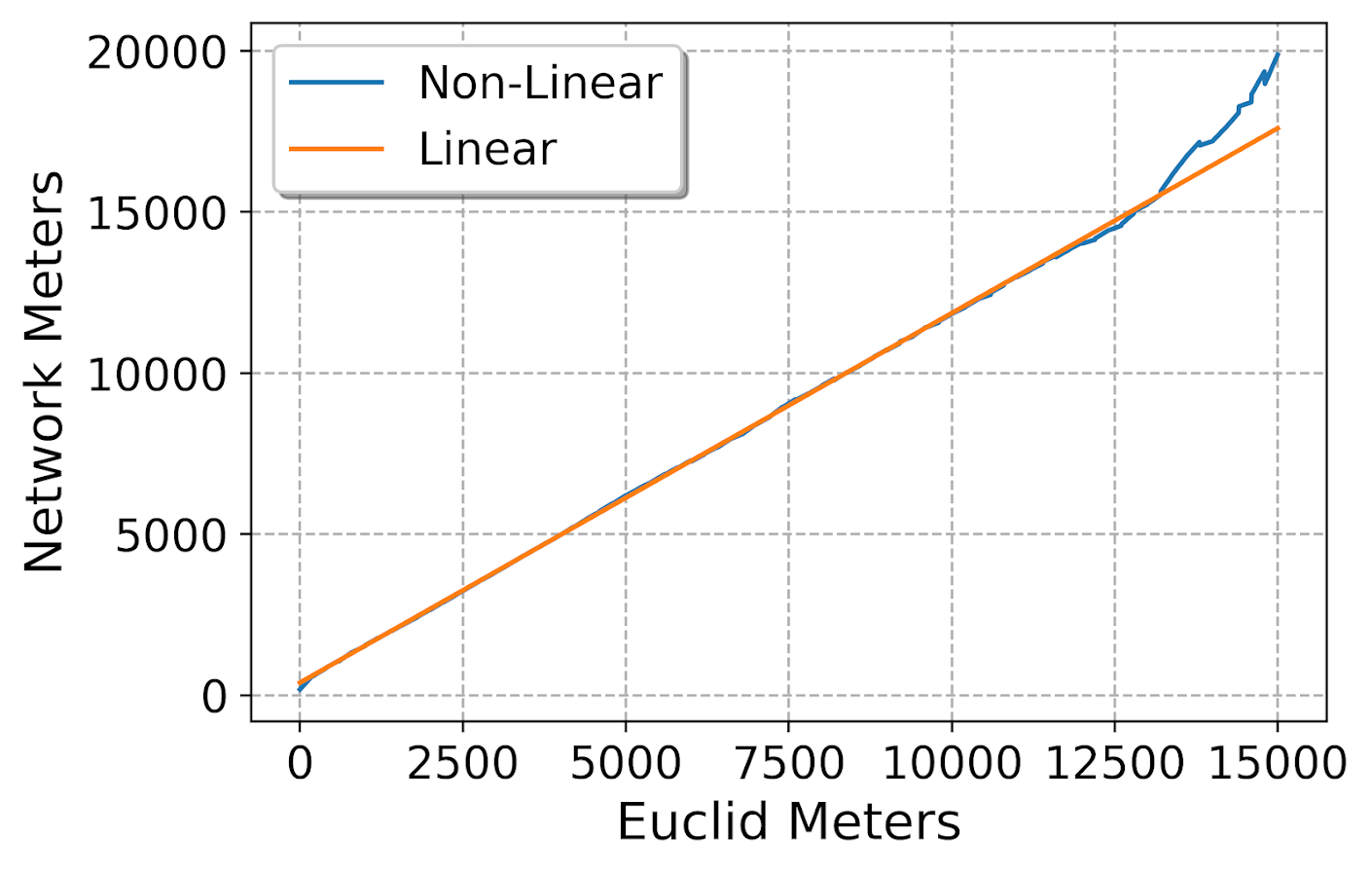Technically the way I have drawn the blue line is misleading, I should have breaks in the line (it is not forced to be connected between bins, like my post on restricted cubic splines is). But I am too lazy to write code to do those splits at the moment.

Now, what does this mean exactly? So for research designs that may want to use network distances and an independent variable, e.g. look at prison visitation as a function of distance, or in my work on patrol redistricting I had to impute some missing travel time distances, these are likely OK to use typical Euclidean distances. Even my paper on survivability for gun shot fatality shows improved accuracy estimates using network distances, but very similar overall effects compared to using Euclidean distances.

So while here I have my computer crunch out the network distances for a day, where the Euclidean distances with the same data only takes a second, e.g. using `scipy.spatial.distance`. So it depends on the nature of the analysis whether that extra effort is worth it. (It helps to have good libraries ease the work, like here I used osmnx for python, and Wouter showed R code using sf to deal with the street networks, hardest part is the networks are often not stored in a way that makes doing the routing very easy. Neither of those libraries were available back in 2014.) Also note you only need to do the network calculations once and then can cache them (and I could have made these network computations go faster if I parallelized the lookup). So it is slightly onerous to do the network computations, but not impossible.

So where might it make a difference? One common use of these network distances in criminology is for analyses like Ripley’s K or near-repeat patterns. I don’t believe using network distances makes a big deal here, but I cannot say for sure. What I believe happens is that using network distances will dilate the distances, e.g. if you conclude two point patterns are clustered starting at 100 meters using Euclidean distances, then if using network it may spread out further and not show clustering until 200 meters. I do not think it would change overall inferences, such as where you make an inference whether two point patterns are clustered or not. (One point is does make a difference is doing spatial permutations in Ripley’s K, you should definitely restrict the simulations to generating hypothetical distributions on the street network and not anywhere in the study area.)

Also Stijn Ruiter makes the point (noted in Wouter’s second post), that street networks may be preferable for prediction purposes. Stijn’s point is related to spatial units of analyses, not to Euclidean vs Network distances. You could have a raster spatial unit of analysis but incorporate street network statistics, and vice-versa could have a vector street unit spatial unit of analysis and use Euclidean distance measures for different measures related to those vector units.

Wouter’s post also brought up another idea I’ve had for awhile, that when using spatial buffers around areas they can be bad control areas, as even if you normalize the area they have a very tiny sliver of network distance attributable to them. I will need to show that for another blog post though. (This was mostly my excuse to learn osmnx to do the routing!)

# Recent Papers on Hot Spots of Crime in Dallas

So I have two different papers that were published recently. Both are on hot spots in Dallas, so might as well discuss them together.

For each I have posted the code to replicate the results (and that spreadsheet has links to preprints as well).

For each as a bit of a background as to the motivation for the projects, Dallas has had official hot spots, named TAAG (Target Area Action Grid). These were clearly larger than what would be considered best practice in identifying hot spots (they were more like entire neighborhoods). I realize ‘best practices’ is a bit wishy-washy, but the TAAG areas ended up covering around 20% of the city (a smidge over 65 square miles). Here is a map of the 2017 areas. There were 54 TAAG areas that covered, so on average each is alittle over 1 square mile.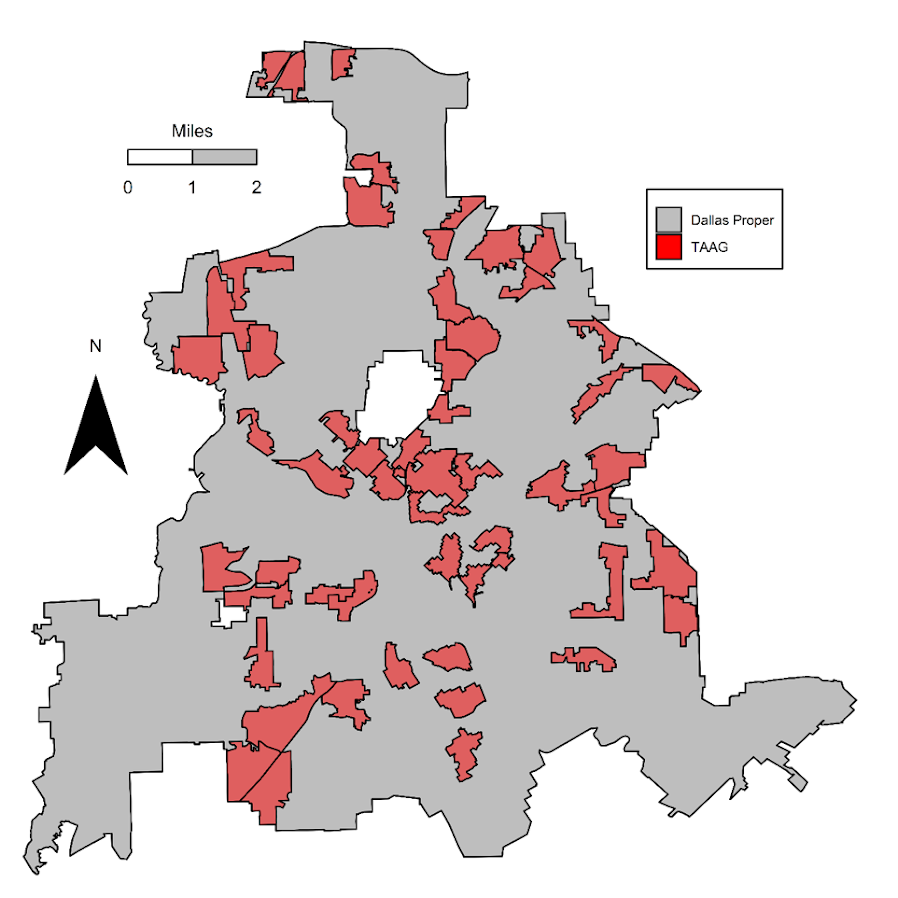Additionally I knew the Dallas police department was interested in purchasing the RTM software to do hot spots. And a separate group, the Dallas Crime Task Force was interested in using the software as well for non-police related interventions.

So I did these projects on my own (with my colleagues Wouter and Sydney of course). It wasn’t paid work for any of these groups (I asked DPD if they were interested, and had shared my results with folks from CPAL before that task force report came out, but nothing much came of it unfortunately). But my results for Dallas data are very likely to generalize to other places, so hopefully they will be helpful to others.

# Machine Learning to Predict and Understand Hot Spots

So I see the appeal for folks who want to use RTM. It is well validated in both theory and practice, and Joel has made a nice software as a service app. But I knew going in that I could likely improve upon the predictions compared to RTM.

RTM tries to find a middle ground between prediction and causality (which isn’t a critique, it is sort of what we are all doing). RTM in the end spits out predictions that are like “Within 800 feet of a Subway Entrances is Risk Factor 1” and “The Density of Bars within 500 Feet is Risk Factor 2”. So it prefers simple models, that have prognostic value for PDS (or other agencies) to identify potential causal reasons for why that location is high crime. And subsequently helps to not only identify where hot spots are, but frame the potential interventions an agency may be interested it.

But this simplicity has a few drawbacks. One is that it is a global model, e.g. “800 feet within a subway entrance” applies to all subway entrances in the city. Most crime generators have a distribution that makes it so most subway entrances are relatively safe, only a few end up being high crime (for an example). Another is that it forces the way that different crime generators predict crime to be a series of step functions, e.g. “within 600 ft” or “a high density within 1000 ft”. In reality, most geographic processes follow a distance decay function. E.g. if you are looking at the relationship between check-cashing stores and street robbery, there are likely to be more very nearby the store, and it tails off in a gradual process the further away you get.

So I fit a more complicated random forest model that has neither of those limitations and can learn much more complicated functions, both in terms of distance to crime generators as well as spatially varying over the city. But because of that you don’t get the simple model interpretation – they are fundamentally conflicting goals. In terms of predictions either my machine learning model or a simpler comparison of using `prior crime = future crime` greatly outperforms RTM for several different predictive metrics.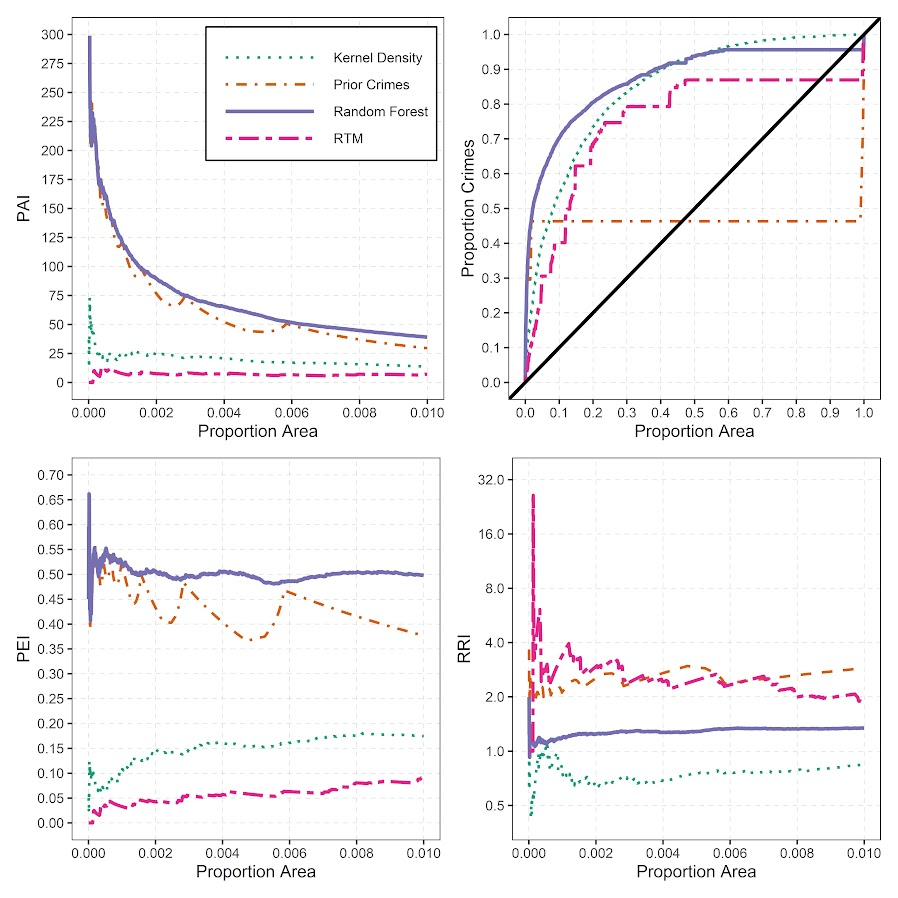So this shows the predictions are better for RTM no matter how you slice the hot spot areas, but again you lose out the prognostic value of RTM. To replace that, I show local interpretability scores for hot spots. I have an online map here for an example. If you click on one of the high crime predicted areas, it gives you a local breakdown of the different variables that contributes to the risk score.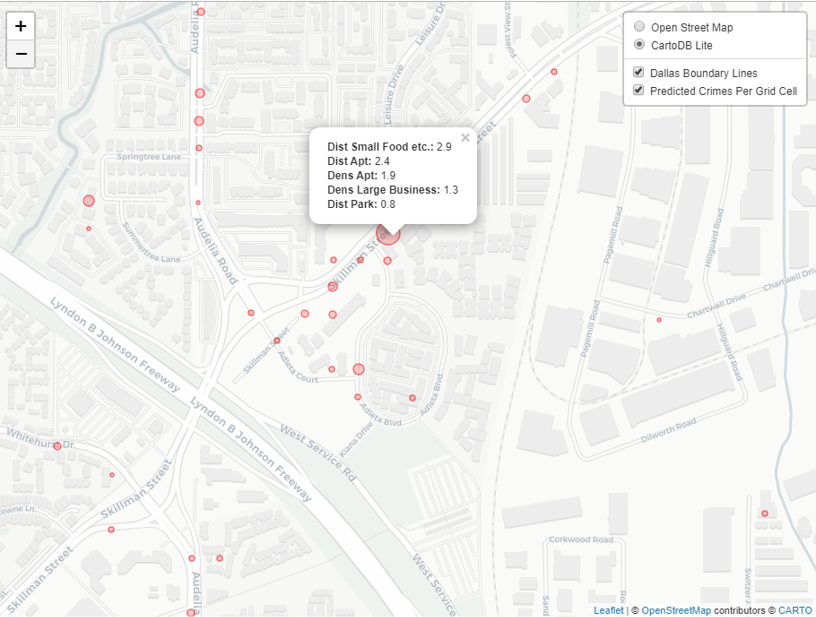So it is still more complicated than RTM, but gets you a local set of factors that potentially contribute to why places are hot spots. (It is still superficial in terms of causality, but PDs aren’t going to be able to get really well identified causal relationships for these types of predictions.)

# Return on Investment for Hot Spots Policing

The second part of this is that Dallas is no doubt in a tight economic bind. And this was even before all the stuff about reforming police budgets. So policing academics have been saying PDs should shift many more resources from reactive to proactive policing for years. But how to make the argument that it is in police departments best interest to shift resources or invest in additional resources?

To do this I aimed to calculate a return on investment on investing in hot spots policing. Priscilla Hunt (from RAND) recently came up with labor cost estimates for crime specifically relevant for police departments. So if an aggravated assault happens PDs (in Texas) typically spend around \$8k in labor costs to respond to the crime and investigate (it is \$125k for a homicide). So based on this, you can say, if I can prevent 10 agg assaults, I then save \$80k in labor costs. I use this logic to estimate a return on investment for PDs to do hot spots policing.

So first I generate hot spots, weighting for the costs of those crimes. Here is an interactive map to check them out, and below is a screenshot of the map.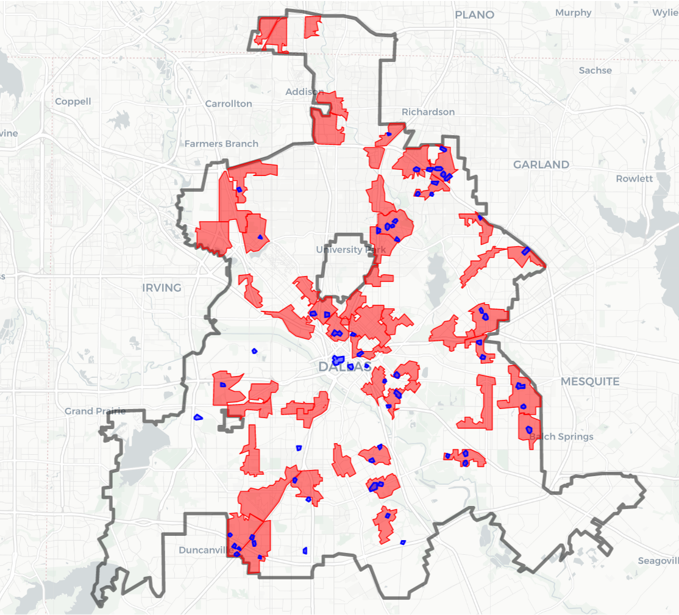I have an example of then calculating a return on investment for the hot spot area that captured the most crime. I get this estimate by transforming meta-analysis estimates of hot spots policing, estimating an average crime reduction, and then backing out how much labor costs that would save a police department. So in this hot spot, an ROI for hot spots policing (for 1.5 years) is \$350k.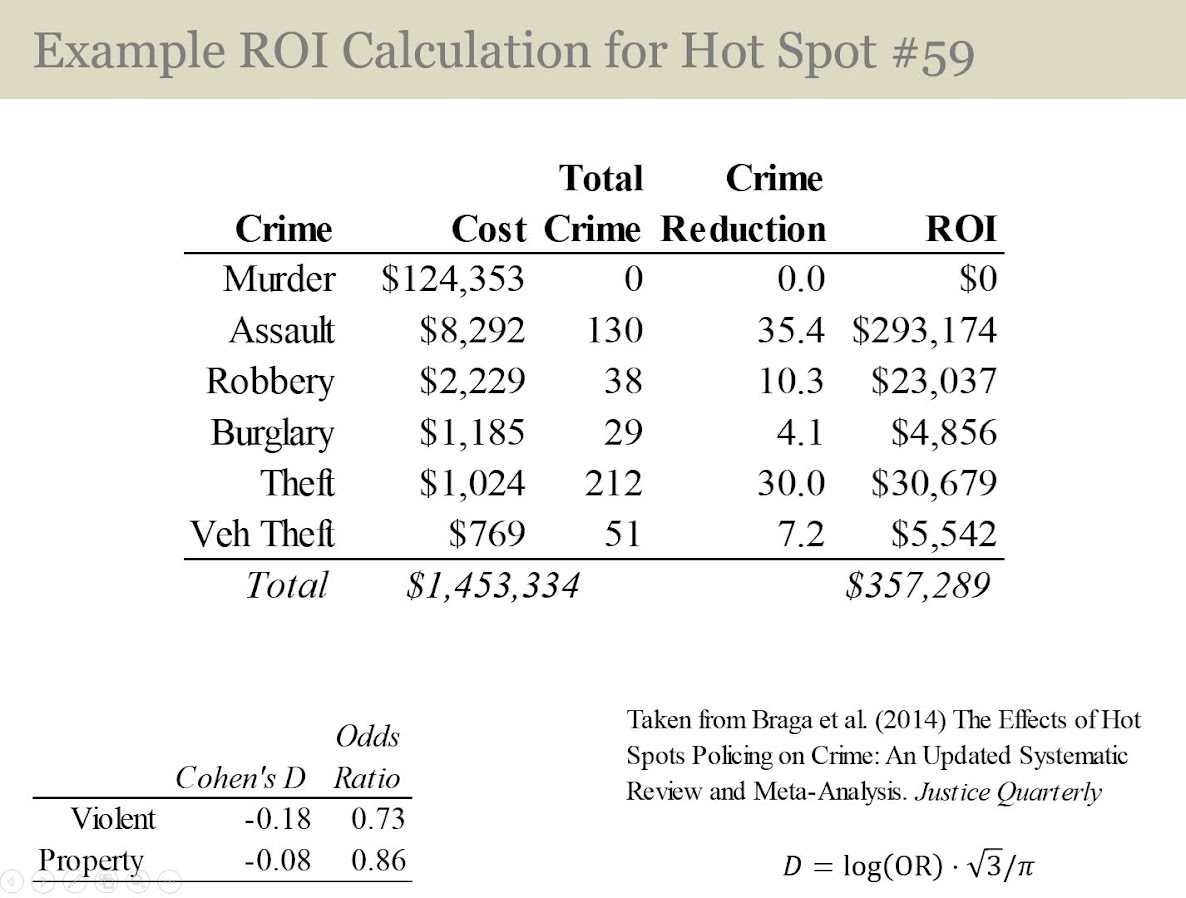That return would justify at least one (probably more like two) full time officers just to be assigned to that specific hot spot. So if you actually hire more officers, it will be around net-zero in terms of labor costs. If you shift around current officers it should be a net gain in labor resources for the PD.

So most of the hot spots I identify in the study if you do this ROI calculation likely aren’t hot enough to justify hot spots policing from this ROI perspective (these would probably never justify intensive overtime that is typical of crackdown like interventions). But a few clearly are, and definitely should be the targets of some type of hot spot intervention.

# A linear programming example for TURF analysis in python

Recently on LinkedIn I saw a very nice example of TURF (Total Unduplicated Reach & Frequency) analysis via Jarlath Quinn. I suggest folks go and watch the video, but for a simple example imagine you are an ice cream food truck, and you only have room in your truck to sell 5 different ice-creams at a time. Some people only like chocolate, others like vanilla and neapolitan, and then others like me like everything. So what are the 5 best flavors to choose to maximize the number of people that like at least one flavor?

You may think this is a bit far-fetched to my usual posts related to criminal justice, but it is very much related to the work I did on identifying optimal gang members to deliver the message in a Focused Deterrence initiative. (I’m wondering if also there is an application in Association Rules/Conjunctive analysis.) But most examples I see of this are in the marketing space, e.g. whether to open a new store, or to carry a new product in a store, or to spend money on ads to reach an audience, etc.

Here I have posted the python code and data used in the analysis, below I go through the steps in formulating different linear programs to tackle this problem. I ended up taking some example simulated data from the XLStat website. (If you have a real data example feel free to share!)

A bit of a side story – growing up in rural Pennsylvania, going out to restaurants was sort of a big event. I specifically remember when we would travel to Williamsport, we would often go to eat at a restaurant called Hoss’s and we would all just order the salad bar buffet. So I am going to pretend this restaurant survey is maximizing the reach for Hoss’s buffet options.

# Frontmatter

Here I am using pulp to fit the linear programming, reading in the data, and I am making up names for the columns for different food items. I have a set of main course meals, sides, and desserts. You will see in a bit how I incorporate this info into the buffet plans.

``````############################################################
#FRONT MATTER

import pandas as pd
import pulp
import os

os.chdir('D:\Dropbox\Dropbox\Documents\BLOG\TURF_Analysis\Analysis')

#This is simulated data from XLStat

#Need 27 total of match up simulated data
main = ['Steak',
'Pizza',
'FriedChicken',
'BBQChicken',
'GrilledSalmon',
'Roast',
'Burger',
'Wings']

'TomatoSoup',
'OnionSoup',
'Peas',
'BrusselSprouts',
'GreenBeans',
'Corn',
'DeviledEggs',
'Pickles']

desserts = ['ChoclateIceCream',
'VanillaIceCream',
'Brownie',
'Blondie',
'CherryPie']

#Renaming columns
surv_data.columns = main + sides + desserts

#Replacing the likert scale data with 0/1
surv_data.replace([1,2,3],0,inplace=True)
surv_data.replace([4,5],1,inplace=True)

#A customer weight example, here setting to 1 for all
surv_data['CustWeight'] = 1
cust_weight = surv_data['CustWeight']
############################################################``````

# Maximizing Customers Reached

And now onto the good stuff, here is an example TURF model linear program. I end up picking the same 5 items that the XLStat program picked in their spreadsheet as well.

``````############################################################

k = 5 #pick 5 items
Cust_Index = surv_data.index
Prod_Index = main + sides + desserts

#Problem and Decision variables
P = pulp.LpProblem("TURF", pulp.LpMaximize)
Cust_Dec = pulp.LpVariable.dicts("Customers Reached", [i for i in Cust_Index], lowBound=0, upBound=1, cat=pulp.LpInteger)
Prod_Dec = pulp.LpVariable.dicts("Products Selected", [j for j in Prod_Index], lowBound=0, upBound=1, cat=pulp.LpInteger)

#Objective Function
P += pulp.lpSum( Cust_Dec[i] * cust_weight[i] for i in Cust_Index )

surv_items = surv_data[Prod_Index] #Dont want the weight variable
#Reached Constraint
for i in Cust_Index:
#Get the products selected
p_sel = surv_items.loc[i] == 1
sel_prod = list(p_sel.index[p_sel])
#Set the constraint
P += Cust_Dec[i] <= pulp.lpSum( Prod_Dec[j] for j in sel_prod )

#Total number of products selected constraint
P += pulp.lpSum( Prod_Dec[j] for j in Prod_Index) == k

#Now solve the model
P.solve()

#Figure out the total reached people
print( pulp.value(P.objective) ) #129

#Print out the products you picked
picked = []
for n,j in enumerate(Prod_Index):
if Prod_Dec[j].varValue == 1:
picked.append( (n+1,j) )

print(picked)

#Same as XLStat
#[(14, 'OnionSoup'), (15, 'Peas'), (16, 'BrusselSprouts'),

#For 5 items, XLStat selected items
# 14 15 16 23 26 that reached 129 people
############################################################``````

One of the things I have done here is to create a ‘weight’ variable associated with each customer. So here I say all of the customers weights are all equal to 1, but you could swap out whatever you wanted. Say you had estimates on how much different individuals spend, so you could give big spenders more weight. (In a criminal justice example, for the Focused Deterrence initiative, folks typically want to target ‘leaders’ more frequently, so you may give them more weight in this example.) Since these examples are based on surveys, you may also want the weight to correspond to the proportion that survey respondent represents in the population, aka raking weights. Or if you have a crazy large survey population, you could use frequency weights for responses that give the exact same picks.

One thing to note as well in this formula is that I recoded the data earlier to be 0/1. You might however consider the likert scale rating 1 to 5 directly, subtract 1 and divide by 4. Then take that weight, and instead of the line:

``Cust_Dec[i] <= pulp.lpSum( Prod_Dec[j] for j in sel_prod )``

You may want something like:

``Cust_Dec[i] <= pulp.lpSum( Prod_Dec[j]*likert_weight[i,j] for j in sel_prod )``

In that case you would want to set the `Cust_i` decision variable to a continuous value, and then maybe cap it at 1 (so you can partially reach customers).

The total number of decision variables will be the number of customers plus the number of potential products, so here only `185 + 27 = 212`. And the number of constraints will be the number of customers plus an additional small number. I’d note you can easily solve systems with 100,000’s of decision variables and constraints on your laptop, so at least for the example TURF analyses I have seen they are definitely within the ‘can solve this in a second on a laptop’ territory.

You can add in additional constraints into this problem. So imagine we always wanted to select one main course, at least two side dishes, and no more than three desserts. Also say you never wanted to pair two items together, say you had two chicken dishes and never wanted both at the same time. Here is how you could do each of those different constraints in the problem.

``````############################################################
#CONSTRAINTS ON ITEMS SELECTED

#Redoing the initial problem, but select 7 items
k = 7
P2 = pulp.LpProblem("TURF", pulp.LpMaximize)
Cust_Dec2 = pulp.LpVariable.dicts("Customers Reached", [i for i in Cust_Index], lowBound=0, upBound=1, cat=pulp.LpInteger)
Prod_Dec2 = pulp.LpVariable.dicts("Products Selected", [j for j in Prod_Index], lowBound=0, upBound=1, cat=pulp.LpInteger)
P2 += pulp.lpSum( Cust_Dec2[i] * cust_weight[i] for i in Cust_Index )
for i in Cust_Index:
p_sel = surv_items.loc[i] == 1
sel_prod = list(p_sel.index[p_sel])
P2 += Cust_Dec2[i] <= pulp.lpSum( Prod_Dec2[j] for j in sel_prod )
P2 += pulp.lpSum( Prod_Dec2[j] for j in Prod_Index) == k

#No Fried and BBQ Chicken at the same time
P2 += pulp.lpSum( Prod_Dec2['FriedChicken'] + Prod_Dec2['BBQChicken']) <= 1
#Exactly one main course
P2 += pulp.lpSum( Prod_Dec2[m] for m in main) == 1
#At least two sides (but could have 0)
P2 += pulp.lpSum( Prod_Dec2[s] for s in sides) >= 2
#No more than 3 desserts
P2 += pulp.lpSum( Prod_Dec2[d] for d in desserts) <= 3

#Now solve the model and print results
P2.solve()
print( pulp.value(P2.objective) ) #137
picked2 = []
for n,j in enumerate(Prod_Index):
if Prod_Dec2[j].varValue == 1:
picked2.append( (n+1,j) )
print(picked2)
#[(10, 'Wings'), (12, 'IcebergSalad'), (14, 'OnionSoup'), (15, 'Peas'),
# (16, 'BrusselSprouts'), (23, 'ChocChipCookie'), (27, 'CherryPie')]
############################################################``````

You could also draw a trade-off curve for how many more people you will reach if you can up the total number of items you can place on the menu, so estimate the model with 4, 5, 6, etc items and see how many more people you can reach if you extend the menu.

One of the other constraints you may consider in this formula is a budget constraint. So imagine instead of the food example, you are working for a marketing company, and you have an advertisement budget. You want to maximize the customer reach given the budget, so here a “product” may be a billboard, radio ad, newspaper ad, etc, but each have different costs. So instead of the constraint `Prod_j == k` where you select so many products, you have the constraint `Prod_j*Cost_j <= Budget`, where each product is associated with a particular cost.

# Alt Formula, Minimizing Cost while Reaching a Set Amount

So in that last bit I mentioned costs for selecting a particular portfolio of products. Another way you may think about the problem is minimizing cost while meeting constraints on the reach (instead of maximizing reach while minimizing cost). So say you were a marketer, and wanted an estimate of how much budget you would need to reach a million people. Or going with our buffet example, imagine we wanted to appeal to at least 50% of our sample (so at least 93 people). Our formula would then be below (where I make up slightly different costs for buffet each of the buffet options).

``````############################################################
#MINIMIZE COST

cost_prod = {'Steak' : 5.0,
'Pizza' : 2.0,
'FriedChicken' : 4.0,
'BBQChicken' : 3.5,
'GrilledSalmon' : 4.5,
'Roast' : 3.9,
'Burger' : 1.5,
'Wings' : 2.4,
'TomatoSoup' : 0.4,
'OnionSoup' : 0.9,
'Peas' : 0.6,
'BrusselSprouts' : 0.5,
'GreenBeans' : 0.4,
'Corn' : 0.3,
'DeviledEggs' : 0.7,
'Pickles' : 0.73,
'ChoclateIceCream' : 1.3,
'VanillaIceCream' : 1.2,
'Brownie' : 1.2,
'Blondie' : 1.3,
'CherryPie' : 1.9}

#Setting up the model with the same selection constraints
n = 100
Pmin = pulp.LpProblem("TURF", pulp.LpMinimize)
Cust_Dec3 = pulp.LpVariable.dicts("Customers Reached", [i for i in Cust_Index], lowBound=0, upBound=1, cat=pulp.LpInteger)
Prod_Dec3 = pulp.LpVariable.dicts("Products Selected", [j for j in Prod_Index], lowBound=0, upBound=1, cat=pulp.LpInteger)
#Minimize this instead of Maximize reach
Pmin += pulp.lpSum( Prod_Dec3[j] * cost_prod[j] for j in Prod_Index )
for i in Cust_Index:
p_sel = surv_items.loc[i] == 1
sel_prod = list(p_sel.index[p_sel])
Pmin += Cust_Dec3[i] <= pulp.lpSum( Prod_Dec3[j] for j in sel_prod )
#Instead of select k items, we want to reach at least n people
Pmin += pulp.lpSum( Cust_Dec3[i]*cust_weight[i] for i in Cust_Index) >= n

#Same constraints on meal choices
Pmin += pulp.lpSum( Prod_Dec3['FriedChicken'] + Prod_Dec3['BBQChicken']) <= 1
Pmin += pulp.lpSum( Prod_Dec3[m] for m in main) == 1
Pmin += pulp.lpSum( Prod_Dec3[s] for s in sides) >= 2
Pmin += pulp.lpSum( Prod_Dec3[d] for d in desserts) <= 3

#Now solve the model and print results
Pmin.solve()

reached = 0
for i in Cust_Index:
reached += Cust_Dec3[i].varValue
print(reached) #100 reached on the nose

picked = []
for n,j in enumerate(Prod_Index):
if Prod_Dec3[j].varValue == 1:
picked.append( (n+1,j,cost_prod[j]) )
cost += cost_prod[j]
print(picked)
#[(9, 'Burger', 1.5), (13, 'TomatoSoup', 0.4), (15, 'Peas', 0.6)]
print(pulp.value(Pmin.objective)) #Total Cost 2.5
############################################################``````

So for this example our minimum budget buffet has some burgers, tomato soup, and peas. (Sounds good to me, I am not a picky eater!)

You can still incorporate all of the same other constraints I discussed before in this formulation. So here we need at a minimum to serve only 3 items to get the (over) 50% reach that we desire. If you wanted fairness type constraints, e.g. you want to reach 60% of females and 40% of males, you could do that as well. In that case you would just have two separate constraints for each group level you wanted to reach (which would also be applicable to the prior maximize reach formula, although you may need to keep upping the number of products selected before you identify a feasible solution).

In the end you could mash up these two formulas into one bi-objective function. You would need to define a term though to balance reach and cost. I’m wondering as well if there is a way to incorporate marginal benefits of sales into this as well, e.g. if you sell a Steak you may make a larger profit than if you sell a Pizza. But I am not 100% sure how to do that in this set up (even though I like all ice-cream, I won’t necessarily buy every flavor if I visit the shop). Similar for marketing adverts some forms may have better reach, but may have worse conversion rates.

# CrimRxiv, Alt-Journal Contributions, and Mike Maltz’s Retrospective

As I’m sure followers of mine know, I am a big proponent of posting pre-prints. Spearheaded by Scott Jacques, he has started a specifically criminology focused pre-print server title CrimRxiv. It is still in beta but anyone can contribute a paper if they want.

One of the things me and Scott have been jamming about is how to leverage crimrxiv to make a journal that not only takes advantage of all the goodies on the internet, such as being able to embed interactive graphics or other rich media directly in a journal articles. But to really widen the scope of what ‘counts’ in terms of scholarly contribution. Why can’t things like a cool app, or a really good video lecture you edited, or a blog post illustrating code be put on the same level with journal articles?

Part of the reason I am writing this blog post is that I saw Michael Maltz recently publish a retrospective on his career on Academia.edu. This isn’t a typical journal article, but despite that there is no reason why you shouldn’t share such pieces. So I was able to convince Mike to post A Retrospective Look at My Professional Life to crimrxiv. When he first posted it on Academia.edu here was my response on how Mike (despite never having crossed paths) has influenced my career.

Hi Michael and thank you for sharing,

I’ve followed your work since a grad student at Albany. I initially got hooked on data viz based on Tufte’s book. When I looked for examples of criminologists discussing data viz you were the only one I found. That was sometime around 2010, so you had that chapter in the handbook of quantitative crim. You also had another article about drawing glyphs to illustrate life course transitions I was familiar with.

When I finished my classes at SUNY, I then worked at Troy as a crime analyst while finishing my dissertation. I doubt any of the coffee shops were the same from your time, but I did like walking over to Famous hotdogs for lunch every now and then.

Most of my work at the PD was making time series graphs and maps. No regression, so most of my stats training was not particularly useful. Even my mapping course I took focused on areal data analysis was not terribly relevant.

I tried to do similar projects to your glyph life-courses with interval censored crime data, but I was never really successful with that, they always ended up being too complicated with even moderately large crime datasets, see https://andrewpwheeler.com/2013/02/28/interval-graph-for-viz-temporal-overlap-in-crime-events/ and https://andrewpwheeler.com/2014/10/02/stacking-intervals/ for my attempts.

What was much more helpful was simply doing monitoring metrics over time, simple running means, and then I just inverted the PDF of the Poisson to give error bars, e.g. https://andrewpwheeler.com/2016/06/23/weekly-and-monthly-graphs-for-monitoring-crime-patterns-spss/. Then cases that were outside the error bands signified an anomalous pattern. In Troy there was an arrest of a single prolific person breaking into cars, and the trend went from a creeping 10 year high to a 10 year low instantly in those graphs.

So there again we have your work on the Poisson distribution and operations research in that JQC article. Also sometime in there I saw a comment you made on Andrew Gelman’s blog pointing to your work with error bands for BJS. Took that ‘fan chart’ idea later on and provided error bands for city level and USA level homicide trends, e.g. https://apwheele.github.io/MathPosts/FanChart_NewOrleans.html. Most of popular discussion of large scale crime trends is misguided over-interpreting short term noise in my opinion.

So all my degrees are in criminal justice, but I have been focusing more on linear programming over time borrowing from operations researchers as well, https://andrewpwheeler.com/2020/05/29/an-intro-to-linear-programming-for-criminologists/. I’ve found that taking outputs from a predictive model and then applying a decision analysis to specifically articulate strategies CJ agencies should take is much more fruitful than the typical way academic research is done.

Thank you again for sharing your story and best, Andy Wheeler

# New paper out: Trauma Center Drive Time Distances and Fatal Outcomes among Gunshot Wound Victims

A recent paper with Gio Circo, Trauma Center Drive Time Distances and Fatal Outcomes among Gunshot Wound Victims, was published in Applied Spatial Analysis and Policy. In this work, me and Gio estimate the marginal effect that drive time distances to the nearest Level 1 trauma center have on the probability a victim dies of a gun shot wound, using open Philadelphia data.

If you do not have access to that published version, here is a pre-print version. (And you can always email me or Gio and ask for a copy.) Also because we use open data, we have posted the data and code used for the analysis. (Gio did most of the work!)

For a bit of the background on the project, Gio had another paper estimating a similar model using Detroit data. But Gio estimated those models with aggregate data. I was familiar with more detailed Philly shooting data, as I used it for an example hot spot cluster map in my GIS crime mapping class.

There are two benefits to leveraging micro data instead of the aggregated data. One is that you can incorporate micro level incident characteristics into the model. The other is that you can get the exact XY coordinates where the incident occurred. And using those exact coordinates we calculate drive time distances to the hospital, which offer a slight benefit in terms of leave-one-out cross-validated accuracy compared to Euclidean distances.

So in terms of incident level characteristics, the biggest factor in determining your probability of death is not the distance to the nearest hospital, but where you physically get shot on your body. Here is a marginal effect plot from our models, showing how the joint effect of injury location (as different colors) and the drive time distance impact the probability of death. So if you get shot in the head vs the torso, you have around a 30% jump in the probability of death from that gun shot wound. Or if you get shot in an extremity you have a very low probability of death as well.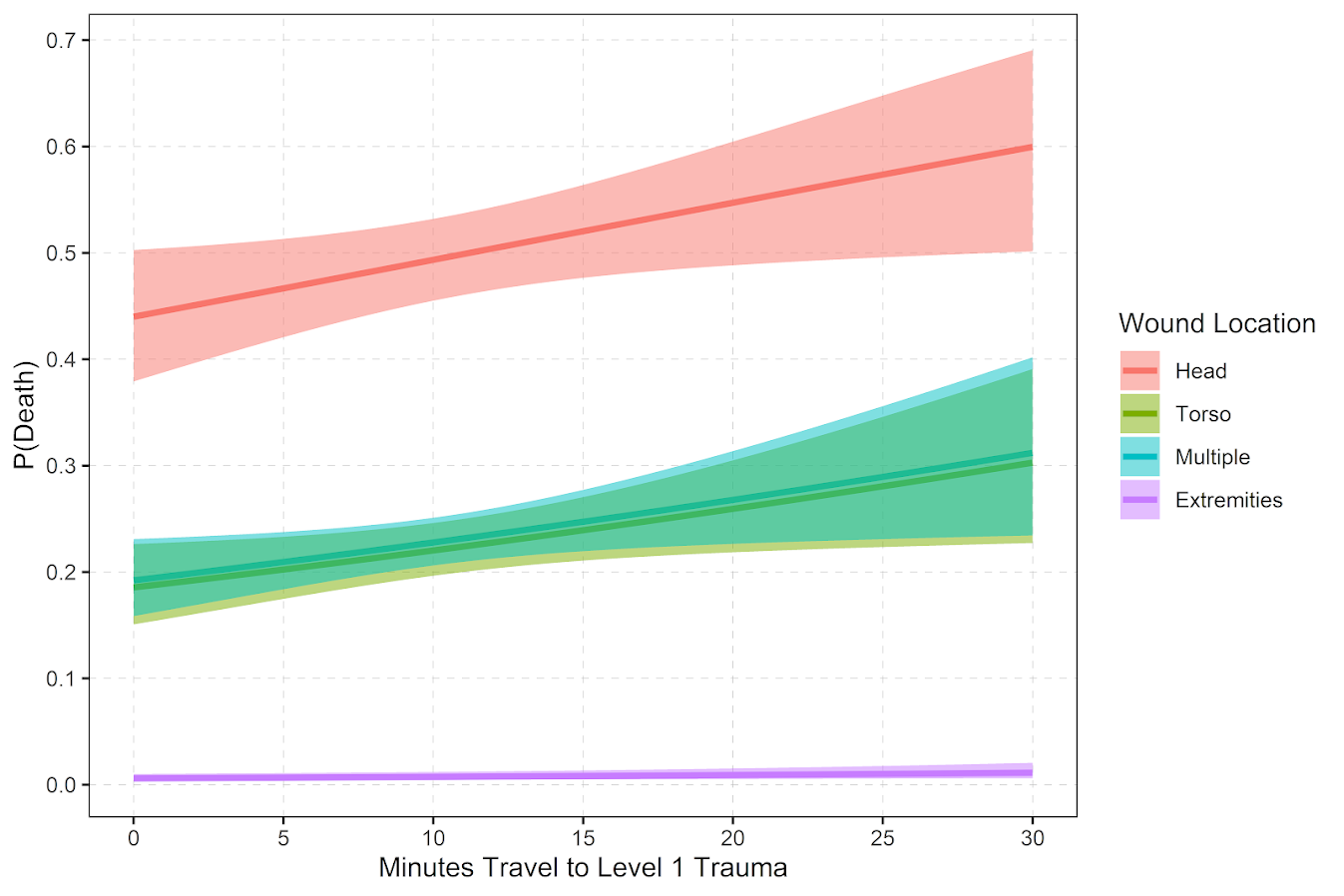But you can see from that the margins for drive times are not negligible. So if you are nearby a hospital and shot in the torso your probability of dying is around 20%, whereas if you are 30 minutes away your probability rises to around 30%. You can then use this to map out isochrone type survivability estimates over the city. This example map is if you get shot in the torso, and the probability of death based on the drive time distance to the nearest Level 1 trauma location.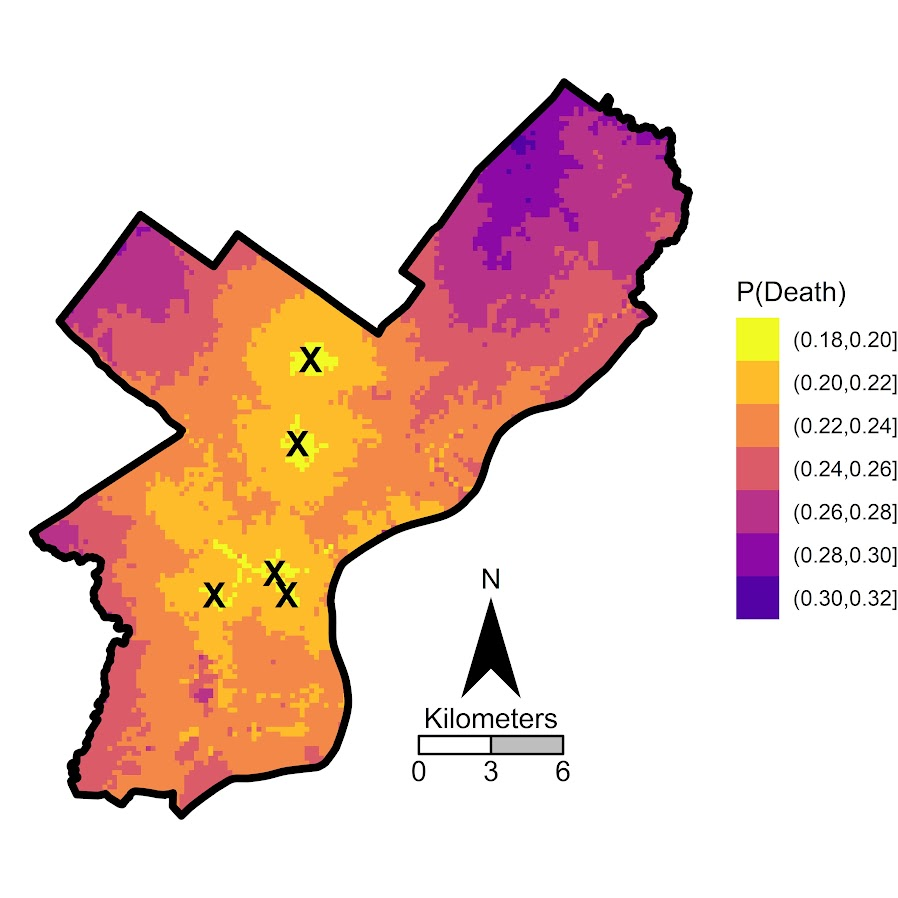Fortunately many shootings do not occur in the northern most parts of Philadelphia, here is a map of the number of shootings over the city for our sample.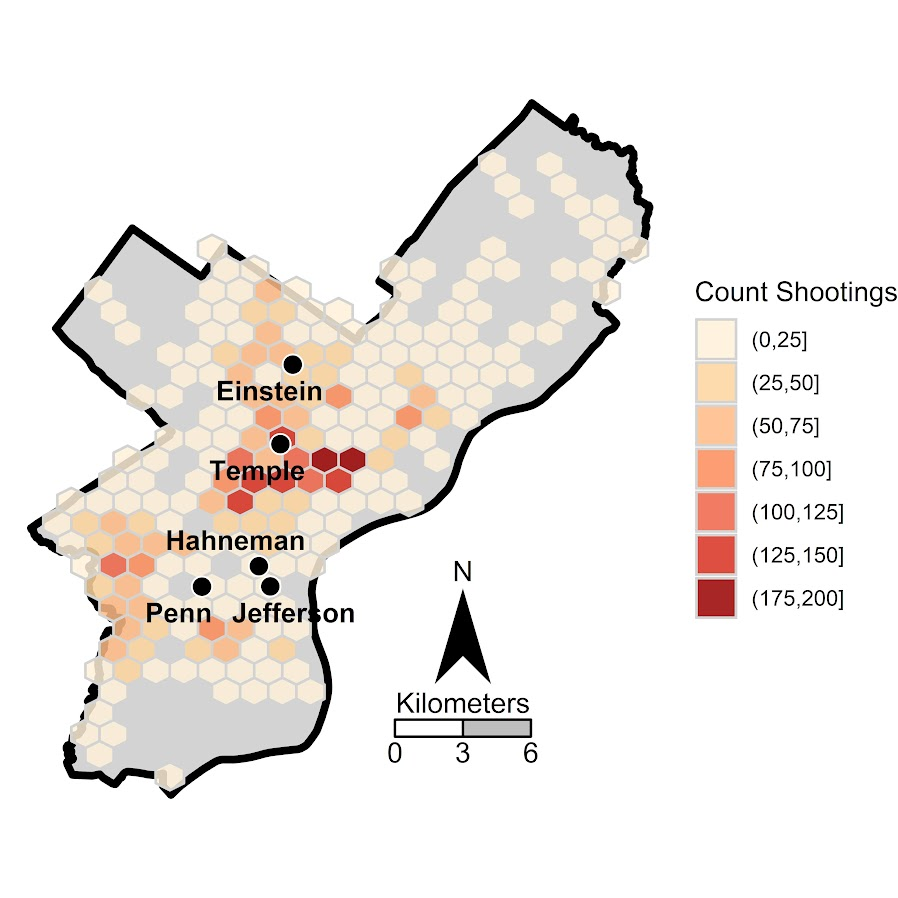You can subsequently use these models to either do hypothetical take a trauma center away or add a trauma center. So given the density of shootings and drive time distances, it might make sense for Philly to invest in a trauma center in the shooting hot spot in the Kensington area (northeast of Temple). (You could technically figure out an ‘optimal’ location given the distribution of shootings, but since you can’t just plop down a hospital wherever it would make more sense to do hypothetical investments in current hospitals.)

For a simplified example, imagine you had 100 shootings in the torso that were an average 20 minutes away. The average probability of death in that case is around 25% (so ~25 homicides). If you hypothetically have a location that is only 5 minutes away, the probability goes down to more like 20% (so ~20 homicides). So in that hypothetical, the distance margin would have prevented 5 deaths.

One future piece of research I would be interested in examining is pre-post Shotspotter. So in that article Jen Doleac is right in that the emipirical evidence for Shotspotter reducing shootings is pretty flimsy, but preventing mortality by getting to the scene faster may be one mechanism that ShotSpotter can justify its cost.

# Discrete time survival models in python

Sorry in the advance for the long post! I’ve wanted to tackle a project on estimating discrete time survival models for awhile now, and may have a relevant project at work where I can use this. So have been crunching out some of this code I am going to share for the last two weeks.

I personally only have one example in my career of estimating discrete time models, I used discrete time models to estimate propensity scores in my demolitions and crime reduction paper (Wheeler et al., 2018), since the demolitions did not occur at all once, but happened over several years. (In that paper I estimated the discrete time models, and then did matches in random cohorts.)

But I was interested in discrete time survival models for one reason – they allow you to estimate very non-linear hazard functions that you cannot with traditional survival models. For Cox models, to do predictions you need to rely on a estimate of the baseline hazard function, and for parametric models (e.g. Weibull) they often can only have monotonic or flat functions (so can’t be low risk and then high risk in a short period). For a good reference about evaluating predictions for survival models, I suggest Haider et al. (2020), and for a general reference for discrete time survival models I suggest the little Sage green book by Paul Allison (Allison, 2014).

For traditional recidivism studies in criminology (e.g. after someone is paroled), I don’t believe the function to be too bumpy like this, so I don’t think prior studies are misleading (e.g. Denver, 2019). But I do think they are worth examining to see if that is the case. For another use case, for chronic offender based police predictions, I think individuals may have more bumpy risk profiles, e.g. you commit a crime and then lay low (so lower risk), or get victimized and may want retaliation (so high risk). A prior work I looked at a year horizon for offender predictions (Wheeler et al., 2019), so I wanted to extend that to shorter time intervals, but never quite got the chance. (Another benefit of discrete time models is that they can incorporate time varying factors no problem the way the model is set up.)

I have code illustrating discrete time models saved on github here. The data I use to illustrate the analysis is taken from Ruderman et al. (2015). This is recidivism for a fairly large cohort. (I don’t think discrete time makes much sense for small samples, you probably need 1000+ to even really consider it I would guess.)

The code ends up being too long to walk though in a blog post. So here are some quick notes/tables/plots, and I encourage you to go check out the github page to dive deeper if you want.

# The Discrete Time Model Setup

The main thing to realize about the discrete time modeling set up is that you just turn your survival data problem into a format you can leverage logistic regression (or whatever binary prediction machine learning model you want). So if we have an original set of survival data that looks like:

``````ID Time Outcome
A   4    1
B   3    0``````

We then explode this dataset into a long format that looks like this:

``````ID Time Outcome
A   1     0
A   2     0
A   3     0
A   4     1
B   1     0
B   2     0
B   3     0``````

So you can see ID A was exploded to 4 observations, and the Outcome variable is only set to 1 at the final time period. For person B, they are exploded 3 observations, but the outcome variable is always set to 0.

Then you model Outcome as a function of time and other covariates, which can be either constant per person or time varying. This then gets you a model that estimates the instant probability of death (or failure) in a particular time sliver. The way I think about it is like this – we can predict whether you will commit a crime sometime within the next week (the cumulative probability over the entire week), or within a particular sliver of time (the probability of committing a crime Friday at 10 pm). Discrete time models pick a sliver of time, e.g. Friday, and calculate the instant probability within that bin.

But then we don’t want to rely on the traditional binary metrics to evaluate this model – we will often want to go from the instant probabilities in a time sliver to the cumulative probabilities. You can take those model estimates though at aggregate them back up to examine over the weekly time horizon example though. So if we have predictions for a new person C that looked like this:

``````ID Time InstantProb
A   1     0.2
A   2     0.1
A   3     0.3
A   4     0.05``````

We could then calculate the cumulative probability of failure over these four time periods. So the failure in time period 1 is just `0.2`. For time period 2 it is `1 - [(1-0.2)*(1-0.1)] = 0.28`. You just then accumulate those individual specific probabilities into cumulative failure probabilities over particular time horizons, which you can then incorporate into cost-benefit analysis for how you will use those predictions in practice. For various metrics we will then examine not just the instant probability our model spits out, but also the cumulative probability of failure.

The main issue with these models is that when exploding the dataset it can result in large samples. So my initial sample of just over 13k observations, when I expand to observed weeks ends up being over 1 million observations. That is not a big deal though, I can still easily do whatever models I want with that data on my personal machine. Probably don’t need to worry about it for most statistical computing projects until maybe you are dealing with over 20 million observations I would bet for most out of the box desktop computers anymore.

# Modeling Notes

In the github page the script `00_PrepData.py` prepares the dataset (transforming to the long format). The original Ruderman data has repeated events, but for simplicity I only take out the first events for individuals, which ends up being just over 13k observations. I then split this into a training dataset and a test dataset, and a set the test dataset to 3k cases.

My temporal unit of analysis I transform into weeks since release, and only examine the discrete time models up to 104 weeks (so two years). Here is a traditional KM plot based on the exploded discrete time training dataset.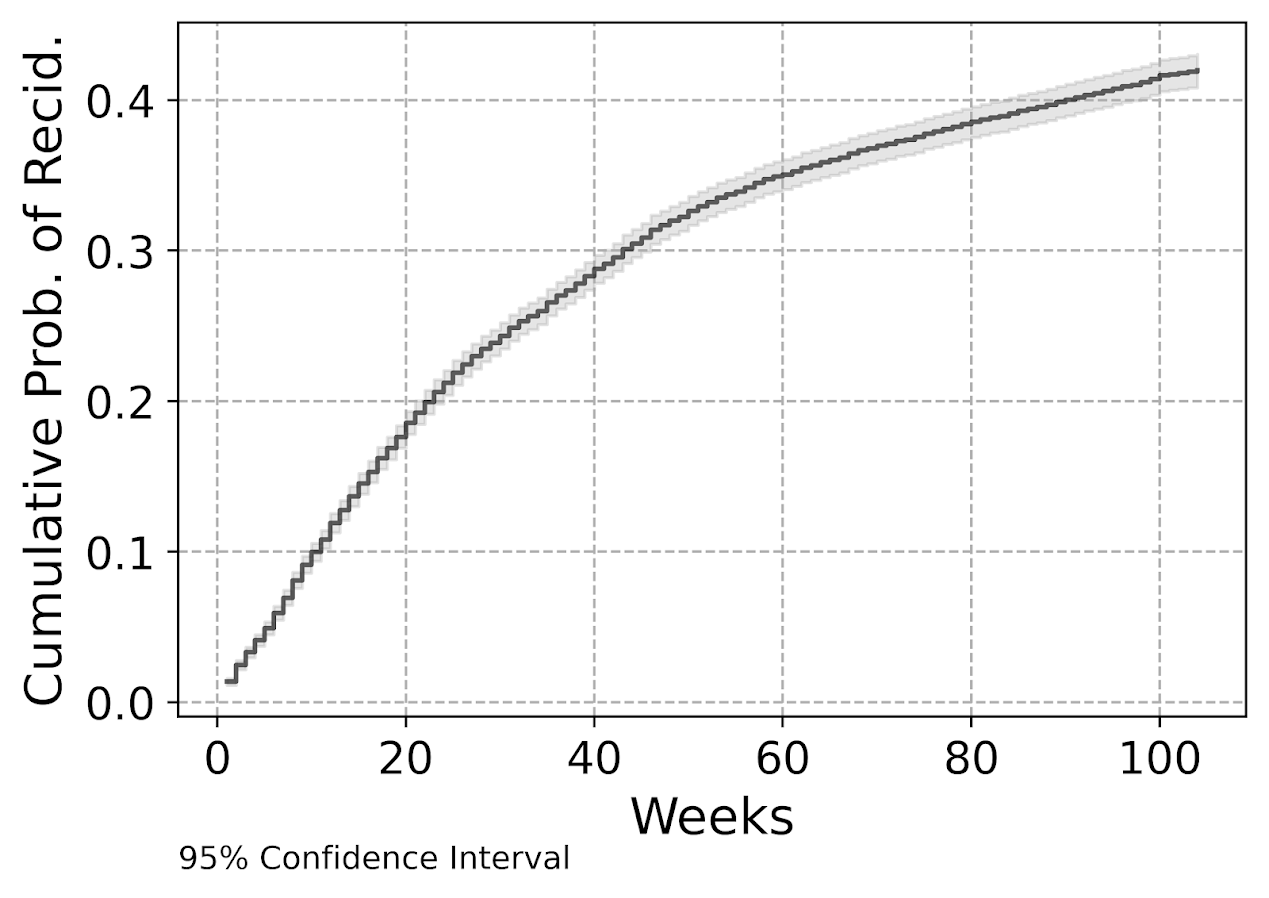But really what we are modeling in this set up is the instant hazard, not the cumulative hazard. So here is a plot of the instant probability of recidivism.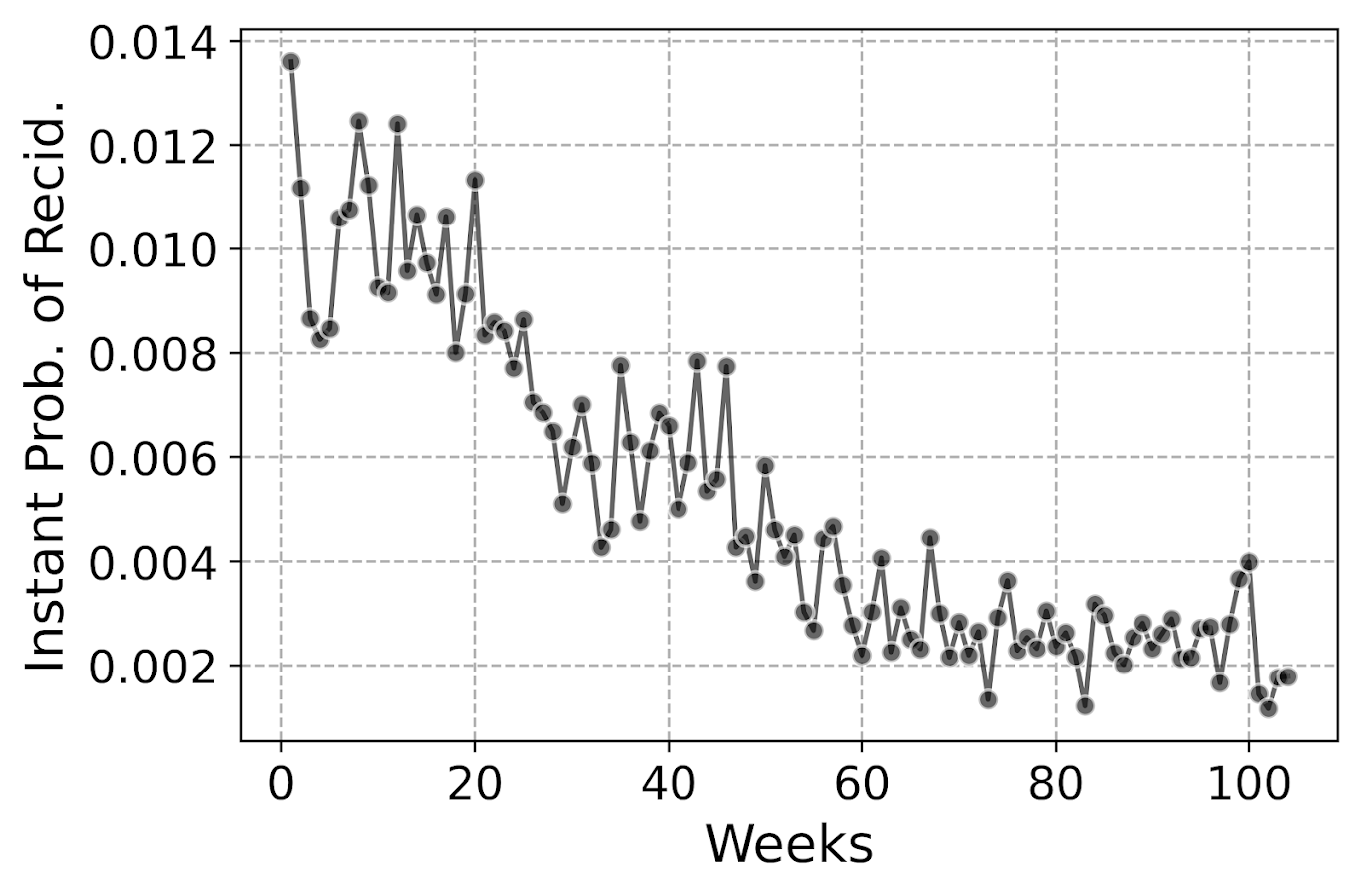You can see that in the first week out, almost 1.4% of the individuals recidivate. There are ups and downs, but the instant probability continues to decrease and slightly flatten out out to 100 weeks. So you can see how over those two years we go from an original dataset of over 10k to around 3k due to censoring.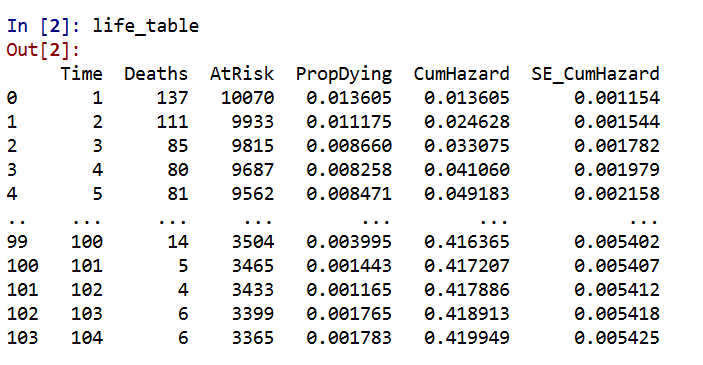Part of the reason I was interested in examining discrete time models is that I was wondering if the instant hazard was bumpy and had some ups and downs when people are first exposed.

But this data appears fairly smooth, so in the end I end up fitting a logistic regression model with restricted cubic splines for time with knot locations at `[4,10,20,40,60,80]`. I also incorporate various interactions with the some of the time invariant covariates in the original Ruderman data (age at first arrest, male, overcrowding, concentrated disadvantage index, and offense category dummies).

I initially tried my GoTo machine learning models of random forests and XGBoost, but they performed quite poorly. Tree based models aren’t very well suited to estimate very tiny probabilities I am afraid. So that will need some more tinkering to see if I can use those machine learning models more effectively in this circumstance. I’m wondering if doing a different loss function makes sense (so do the loss based on the cumulative hazard instead of the instant). Here also I did not regularize the logit model, but with time varying factors that may make sense.

The Haider paper looks at the R MLTR package, which is similar to here but slightly different, in that they are modeling the cumulative hazard directly instead of the instant hazard. (So instead of chopping off the 1’s and the end of the vector, you keep padding them on for observations.) So in that case you want to enforce monotonic constraints on the time effect.

# Checking Out Individual Predictions

The remaining sections in the blog post are all taken from the second `01_EvalTime.py` script. So first, after you generate your predictions on the training data, you can then pull out a particular individual and check out our predictions for their cumulative survival probability based on our predictive model. The red line shows that this individual actually recidivated at 45 weeks, in which their cumulative risk was just above 20%.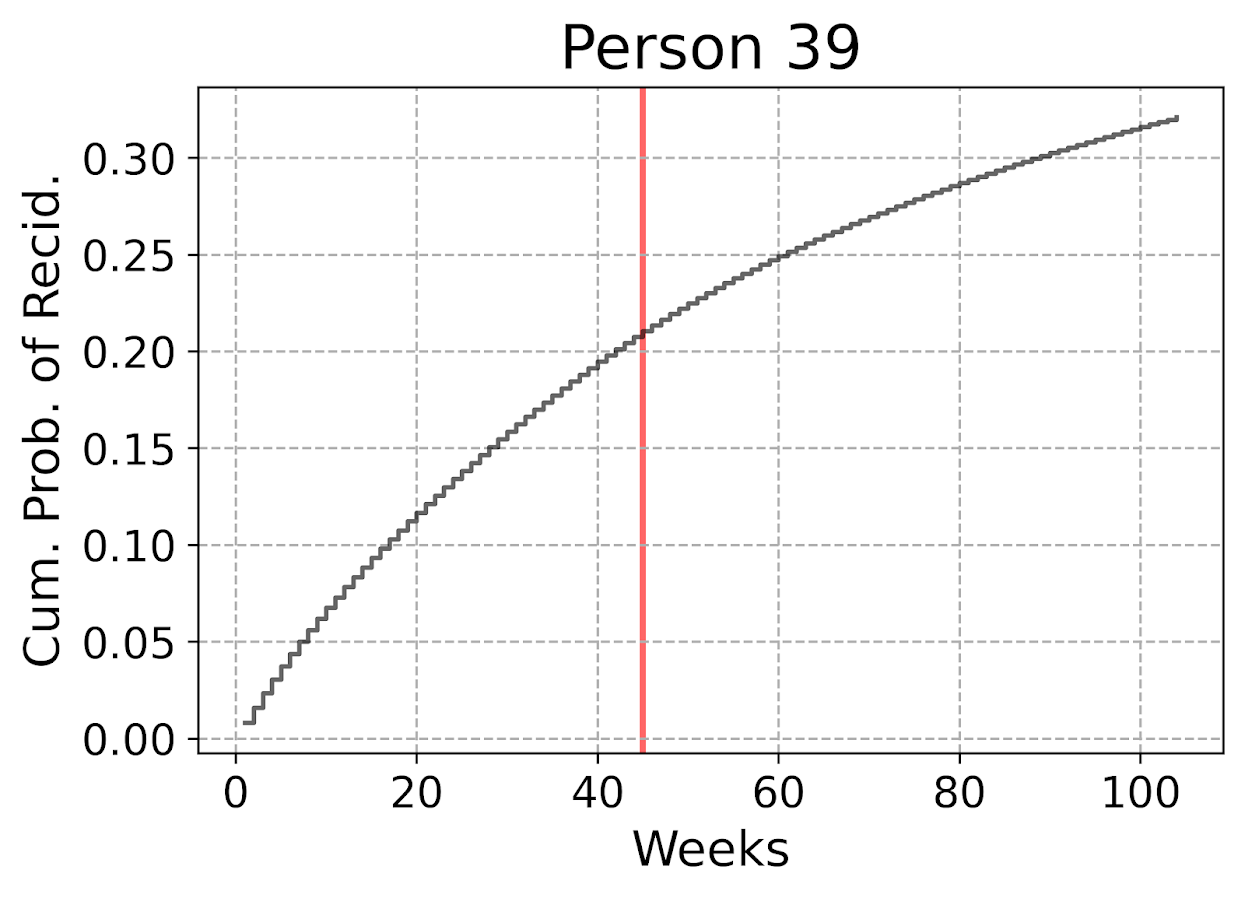The cumulative probability will never be super interesting though – in that even if you had a very wiggly instant hazard the cumulative hazard is always monotonically increasing. So if you check out the instant hazard this will show how a persons risk level varies over time.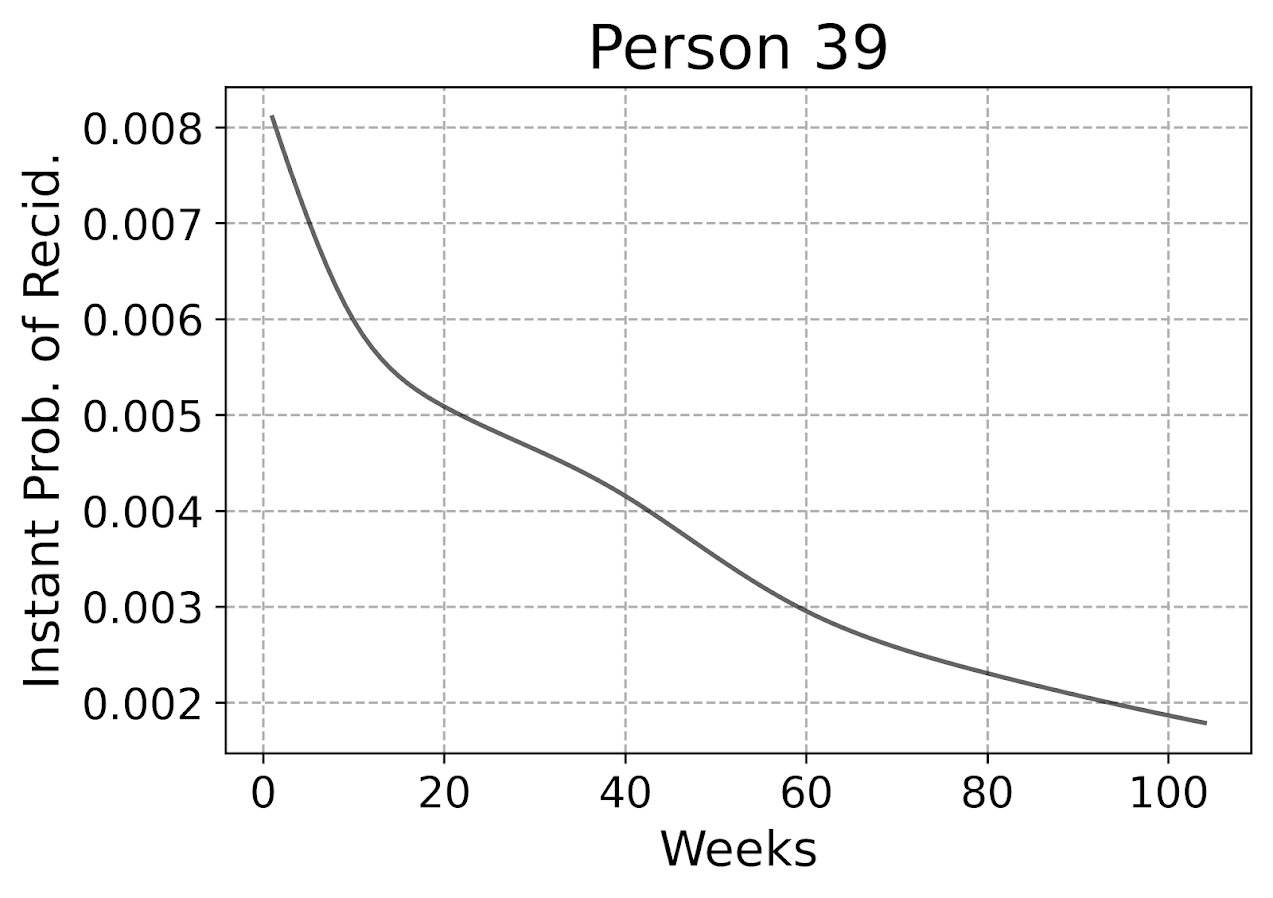So we can see here that person 39 had a predicted high risk when they are first released, but gradual decreases in a few steps over time. The way I have modeled this using restricted cubic splines it has to be smooth, but you could say incorporate dummy variables for the first 10 weeks, in which case this prediction could be quite bumpy.

Given this always shows monotonically decreasing hazard, you wouldn’t be able to exactly fit that function using parametric models, but they would be not too far off. So this dataset doesn’t appear to be a real great showcase of the utility of discrete time models!

But doing some plots of the instant hazards may be interesting to try to identify particular different risk profiles, or maybe even use some clustering (like group based trajectory models) to identify particular latent risk profiles. (It may be most people are smoothly decreasing, but some people have bumpier profiles.)

# Evaluating Model Calibration

Haider et al. (2020) break down predictive metrics to evaluate survival models into two types: calibration is that the model predictions match actual cases, e.g. if my model says the probability of failure is 20%, does the data actually show failure in 20% of the cases. The other is discrimination, can I rank individuals as high risk to low risk, and do the high risk ones have the negative outcome more frequently.

While the Haider paper has various metrics, I am kind of confused how to do them in practice. My confusion mostly stems from the test dataset will ultimately have censoring in it as well, so the calibration metrics need to take this into account. Here are my attempts at a few plots that take on the task of checking model calibration.

First, I’ve previously discussed what I call a lift calibration chart. I adjust it here though to account for the fact that we have interval censoring, and I create ignorance bounds for the actual proportion of failures in the dataset.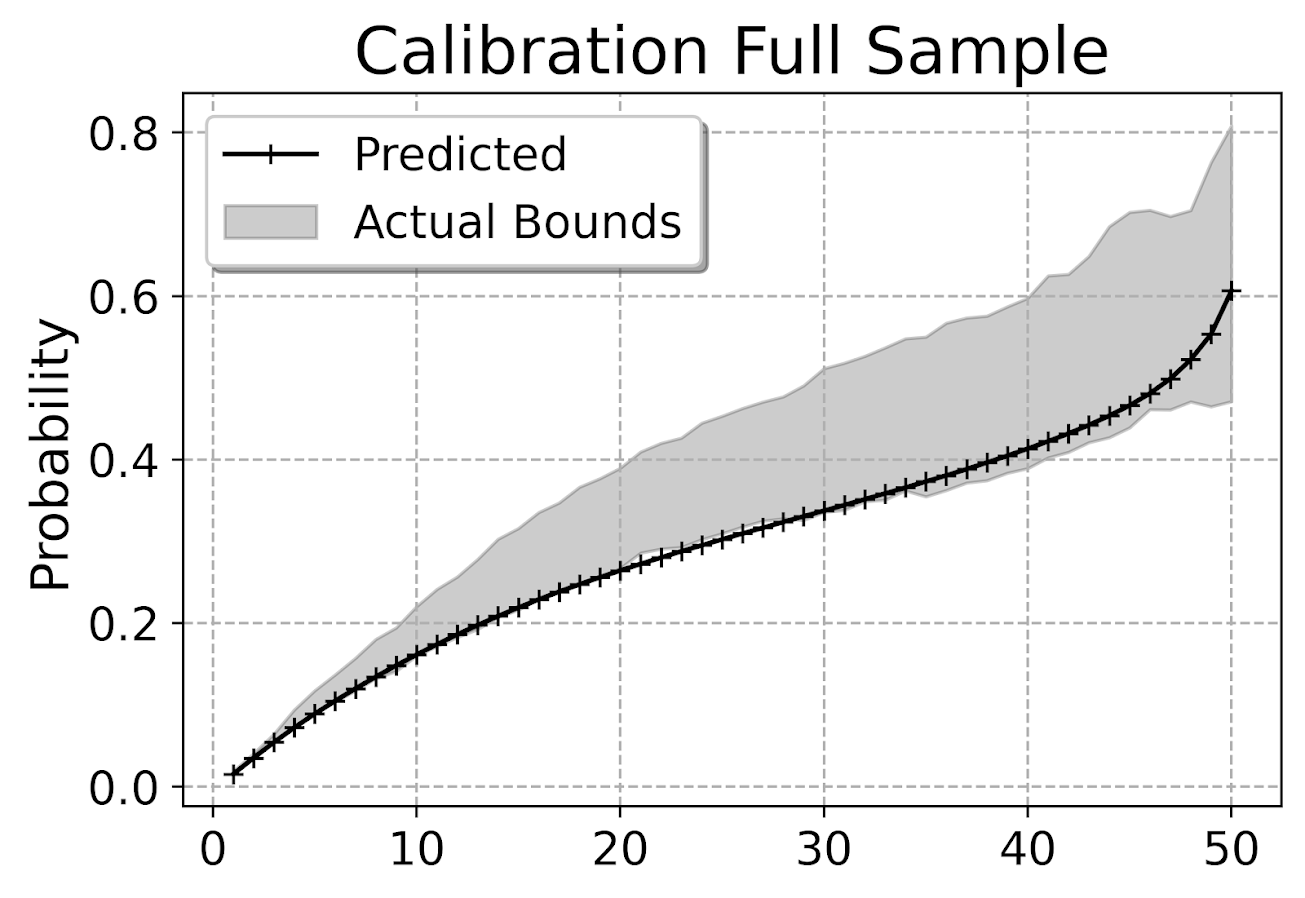This is for the full sample, which I expanded out and did calculations for up to 104 weeks for everyone. You can do a slice of the data though for a particular time period and check the same calibration. So here is an example checking calibration at one year out.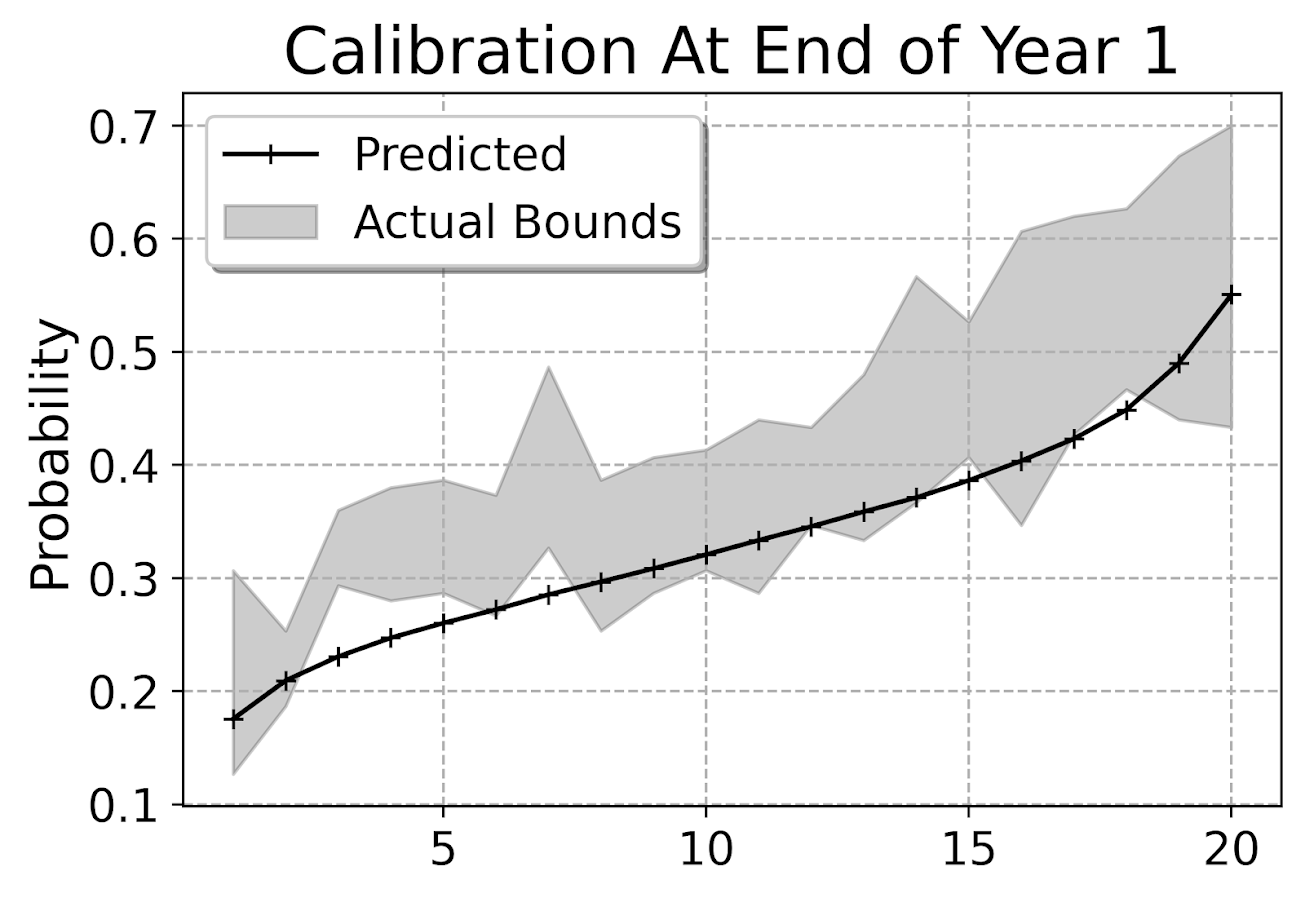The earlier in time the smaller the ignorance bands will be (as there will be less censoring in sample). Here is what the created dataset looks like to illustrate how the ignorance bands are calculated.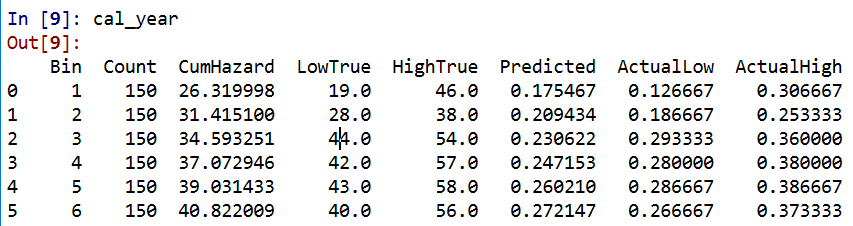The `CumHazard` column is my predicted line, which I break down into 20 bins for that yearly plot (so with 3000 training dataset observations, results in bins of 150 observations). Then you can see the `LowTrue` column (in Bin 1) signifies I observed 19 failures in that set of observations, but there ending up being a total of 27 observations censored in that bin, `46 - 19`. So the actual proportion in the data could either be 19/150 (all censored never recidivate) or 46/150 (all of those who were censored would end up recidivating). I would suggest for notes on ignorance bounds like these (which also apply to ECDF type functions), Ferson et al. (2007).

I’d note that this is the same way you generate data for a Hosmer-Lemeshow test for logit models, but I don’t bother with the Chi-Square test. For large samples it will always reject, and small samples it may mean you just have low power, not that your model is well calibrated. So doing that stat test is a lose-lose IMO. But you can just make the plot to see whether your predictions are on the mark, or if they are low or high on average. Here we can see that they hug the lower ignorance band, so are not too bad. But may be a shade too low (more people recidivate than predicted).

This calibration is examining the probability, but another way to think about calibration here is calibrated in terms of time, e.g. I say something will happen in 30 weeks, does it actually happen in 30 weeks? Here is my attempt at a plot to check that out. Using the test dataset, I generate the usual KM estimate. Then based on the predicted probabilites, I generate simulated outcomes for individuals (here 99), and then plot the range of those outcomes on the same chart.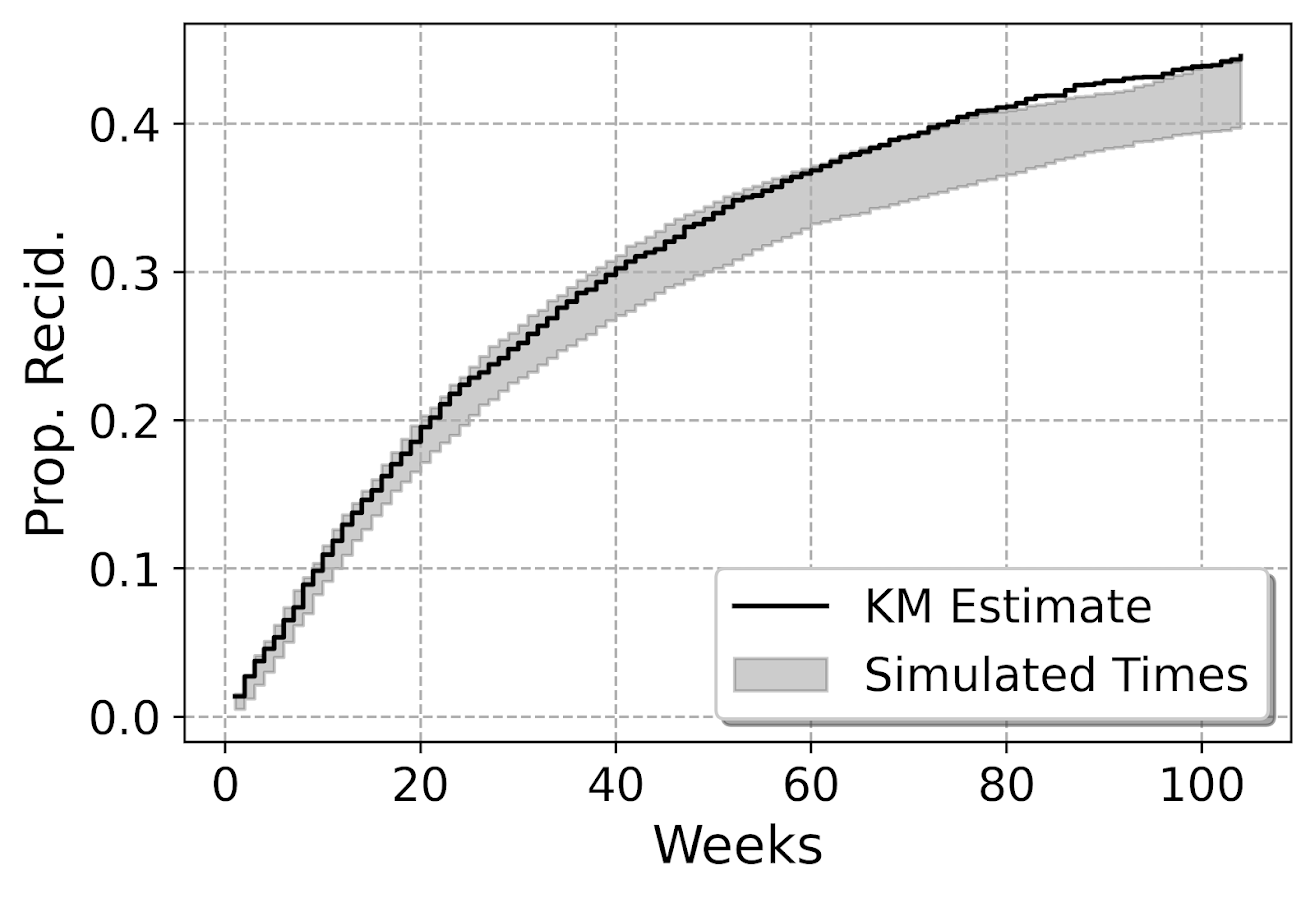So here you can see that my predicted failure times are somewhat longer than observed in the data (simulation bands slightly below observed for the later time periods). These two charts are likely not in contradiction, the error bands in each show somewhat observe patterns, so they both hint at my model is conservative in assigning risk. But it is not too shabby in terms of calibration (you should have seen some of these plots when I was trying random forest and XGBoost models!).

I’m wondering offhand if I have some edge effects going on. So maybe even if I am only interested in examining a time horizon of two years, I should still tack on longer time periods for the initial models.

Both of these charts you can subset the data and look at the same chart, so here is an example table generated for simulations based on 332 test dataset females. Because the sample is smaller, the simulated bands are wider, so the observed KM cumulative hazard estimate appears well inside the bands here for the female subsample. (Probably because of less diagnostic ability to identify tiny bits off in the calibration.)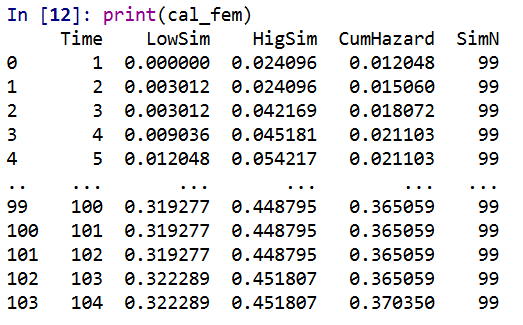# Evaluating Model Discrimination

The second way you might evaluate survival predictions is in terms of rankings, can I discriminate in my model between individuals who are high risk and who are low risk. One of the crazy things about these individual level survival curves is that they can cross! So imagine we had a set of two individuals and are looking at a horizon of four periods:

``````ID Time InstProb CumProb
A   1      0.1     0.1
A   2      0.1     0.19
A   3      0.1     0.271
A   4      0.1     0.3439

B   1      0.2     0.2
B   2      0.1     0.28
B   3      0.05    0.316
B   4      0.01    0.32284``````

So person B is at higher risk right away. So if we ranked these individuals for who was more likely to recidivate, ID B will be ranked higher for periods 1, 2 and 3. But by period 4, ID A is at higher risk in terms of their cumulative probability of recidivating.

The simplest metric to evaluate discrimination IMO is AUC (which is related to the concordance metric). And to do that you just do slices of particular weeks, and then calculate the AUC based on the cumulative failure probability estimate at that time period.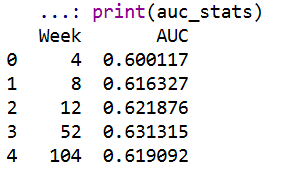So you can see here that it is pretty meh – only AUC stats around 0.6 for my logit model. So better than the random 0.5, but not by much. Even though my model appears to be reasonably calibrated, it is nothing to brag to grandma about being able to identify people at different risk levels for recidivism, not matter the time horizon I am interested in.

For this estimate I just dropped censored observations, so I am not sure how to deal with them in this case. If you have suggestions or references let me know! But offhand I don’t think they are too off, the earlier time periods should have less censoring, but they are all pretty close in terms of the overall metric.

# Future Stuff?

Besides seeing how others have dealt with censoring in their prediction metrics, another metric introduced in the Haider et al. (2020) paper is a Brier Score that is both a calibration and discrimination metric.

Also for folks interested in survival analysis in python, I suggest to check out statsmodel or the lifelines packages.

# Making smoothed scatterplots in python

The other day I made a blog post on my notes on making scatterplots in matplotlib. One big chunk of why you want to make scatterplots though is if you are interested in a predictive relationship. Typically you want to look at the conditional value of the Y variable based on the X variable. Here are some example exploratory data analysis plots to accomplish that task in python.

I have posted the code to follow along on github here, in particular `smooth.py` has the functions of interest, and below I have various examples (that are saved in the `Examples_Conditional.py` file).

# Data Prep

First to get started, I am importing my libraries and loading up some of the data from my dissertation on crime in DC at street units. My functions are in the `smooth` set of code. Also I change the default matplotlib theme using `smooth.change_theme()`. Only difference from my prior posts is I don’t have gridlines by default here (they can be a bit busy).

``````#################################
import pandas as pd
import numpy as np
import matplotlib.pyplot as plt
import seaborn as sns
import statsmodels.api as sm
import os
import sys

mydir = r'D:\Dropbox\Dropbox\PublicCode_Git\Blog_Code\Python\Smooth'
data_loc = r'https://dl.dropbox.com/s/79ma3ldoup1bkw6/DC_CrimeData.csv?dl=0'
os.chdir(mydir)

#My functions
sys.path.append(mydir)
import smooth
smooth.change_theme()

#Dissertation dataset, can read from dropbox
#################################``````

# Binned Conditional Plots

The first set of examples, I bin the data and estimate the conditional means and standard deviations. So here in this example I estimate `E[Y | X = 0]`, `E[Y | X = 1]`, etc, where Y is the total number of part 1 crimes and x is the total number of alcohol licenses on the street unit (e.g. bars, liquor stores, or conv. stores that sell beer).

The function name is `mean_spike`, and you pass in at a minimum the dataframe, x variable, and y variable. I by default plot the spikes as +/- 2 standard deviations, but you can set it via the `mult` argument.

``````####################
#Example binning and making mean/std dev spike plots

smooth.mean_spike(DC_crime,'TotalLic','TotalCrime')

mean_lic = smooth.mean_spike(DC_crime,'TotalLic','TotalCrime',
plot=False,ret_data=True)
####################``````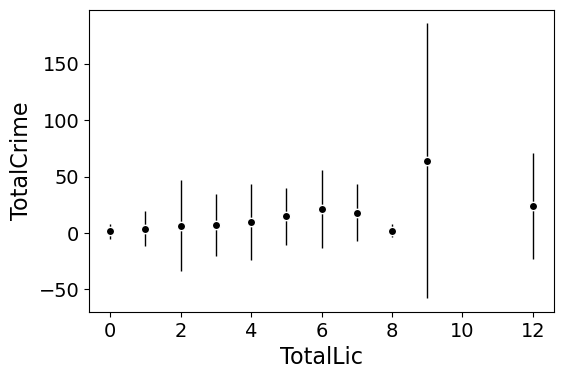This example works out because licenses are just whole numbers, so it can be binned. You can pass in any X variable that can be binned in the end. So you could pass in a string for the X variable. If you don’t like the resulting format of the plot though, you can just pass `plot=False,ret_data=True` for arguments, and you get the aggregated data that I use to build the plots in the end.

``````mean_lic = smooth.mean_spike(DC_crime,'TotalLic','TotalCrime',
plot=False,ret_data=True)``````

Another example I am frequently interested in is proportions and confidence intervals. Here it uses exact binomial confidence intervals at the 99% confidence level. Here I clip the burglary data to 0/1 values and then estimate proportions.

``````####################
#Example with proportion confidence interval spike plots

DC_crime['BurgClip'] = DC_crime['OffN3'].clip(0,1)
smooth.prop_spike(DC_crime,'TotalLic','BurgClip')

####################``````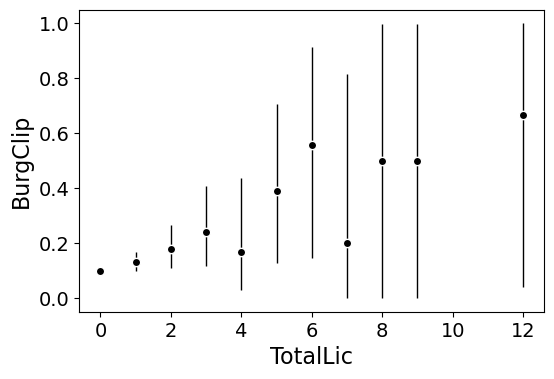A few things to note about this is I clip out bins with only 1 observation in them for both of these plots. I also do not have an argument to save the plot. This is because I typically only use these for exploratory data analysis, it is pretty rare I use these plots in a final presentation or paper.

I will need to update these in the future to jitter the data slightly to be able to superimpose the original data observations. The next plots are a bit easier to show that though.

# Restricted Cubic Spline Plots

Binning like I did prior works out well when you have only a few bins of data. If you have continuous inputs though it is tougher. In that case, typically what I want to do is estimate a functional relationship in a regression equation, e.g. `Y ~ f(x)`, where `f(x)` is pretty flexible to identify potential non-linear relationships.

Many analysts are taught the loess linear smoother for this. But I do not like loess very much, it is often both locally too wiggly and globally too smooth in my experience, and the weighting function has no really good default.

Another popular choice is to use generalized additive model smoothers. My experience with these (in R) is better than loess, but they IMO tend to be too aggressive, and identify overly complicated functions by default.

My favorite approach to this is actually then from Frank Harrell’s regression modeling strategies. Just pick a regular set of restricted cubic splines along your data. It is arbitrary where to set the knot locations for the splines, but my experience is they are very robust (so chaning the knot locations only tends to change the estimated function form by a tiny bit).

I have class notes on restricted cubic splines I think are a nice introduction. First, I am going to make the same dataset from my class notes, the US violent crime rate from 85 through 2010.

``````years = pd.Series(list(range(26)))
vcr = [1881.3,
1995.2,
2036.1,
2217.6,
2299.9,
2383.6,
2318.2,
2163.7,
2089.8,
1860.9,
1557.8,
1344.2,
1268.4,
1167.4,
1062.6,
945.2,
927.5,
789.6,
734.1,
687.4,
673.1,
637.9,
613.8,
580.3,
551.8,
593.1]

yr_df = pd.DataFrame(zip(years,years+1985,vcr), columns=['y1','years','vcr'])``````

I have a function that allows you to append the spline basis to a dataframe. If you don’t pass in a `data` argument, in returns a dataframe of the basis functions.

``````#Can append rcs basis to dataframe
kn = [3.0,7.0,12.0,21.0]
smooth.rcs(years,knots=kn,stub='S',data=yr_df)``````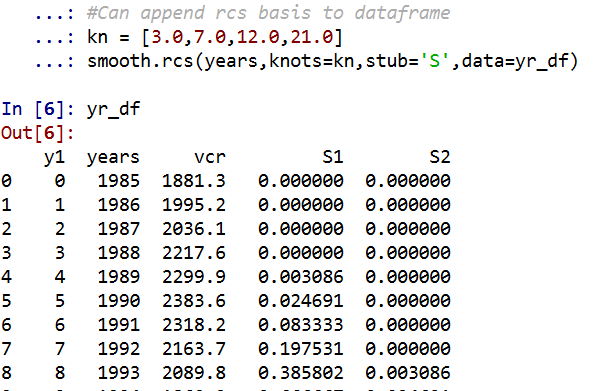I also have in the code set Harrell’s suggested knot locations for the data. This ranges from 3 to 7 knots (it will through an error if you pass a number not in that range). This here suggests the locations `[1.25, 8.75, 16.25, 23.75]`.

``````#If you want to use Harrell's rules to suggest knot locations
smooth.sug_knots(years,4)``````

Note if you have integer data here these rules don’t work out so well (can have redundant suggested knot locations). So Harell’s defaults don’t work with my alcohol license data. But it is one of the reasons I like these though, I just pick regular locations along the X data and they tend to work well. So here is a regression plot passing in those knot locations `kn = [3.0,7.0,12.0,21.0]` I defined a few paragraphs ago, and the plot does a few vertical guides to show the knot locations.

``````#RCS plot
smooth.plot_rcs(yr_df,'y1','vcr',knots=kn)``````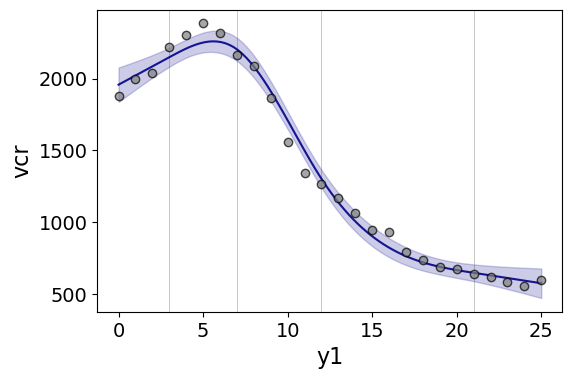Note that the error bands in the plot are confidence intervals around the mean, not prediction intervals. One of the nice things though about this under the hood, I used statsmodels glm interface, so if you want you can change the underlying link function to Poisson (I am going back to my DC crime data here), you just pass it in the `fam` argument:

``````#Can pass in a family argument for logit/Poisson models
smooth.plot_rcs(DC_crime,'TotalLic','TotalCrime', knots=[3,7,10,15],
fam=sm.families.Poisson(), marker_size=12)``````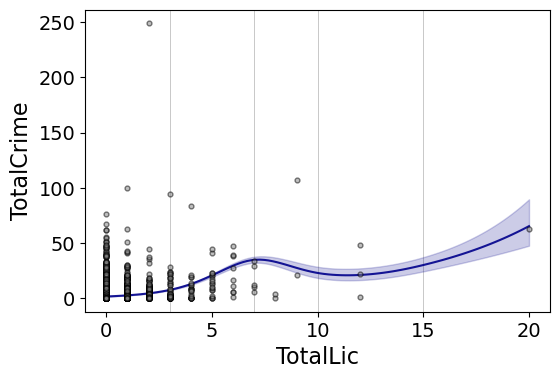This is a really great example for the utility of splines. I will show later, but a linear Poisson model for the alcohol license effect extrapolates very poorly and ends up being explosive. Here though the larger values the conditional effect fits right into the observed data. (And I swear I did not fiddle with the knot locations, there are just what I picked out offhand to spread them out on the X axis.)

And if you want to do a logistic regression:

``````smooth.plot_rcs(DC_crime,'TotalLic','BurgClip', knots=[3,7,10,15],
fam=sm.families.Binomial(),marker_alpha=0)``````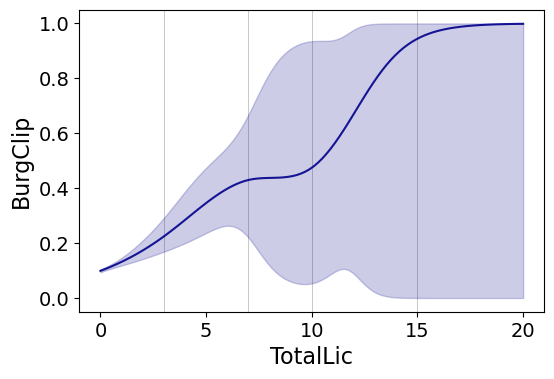I’m not sure how to do this in a way you can get prediction intervals (I know how to do it for Gaussian models, but not for the other glm families, prediction intervals probably don’t make sense for binomial data anyway). But one thing I could expand on in the future is to do quantile regression instead of glm models.

# Smooth Plots by Group

Sometimes you want to do the smoothed regression plots with interactions per groups. I have two helper functions to do this. One is `group_rcs_plot`. Here I use the good old iris data to illustrate, which I will explain why in a second.

``````#Superimposing rcs on the same plot
smooth.group_rcs_plot(iris,'sepal_length','sepal_width',
'species',colors=None,num_knots=3)``````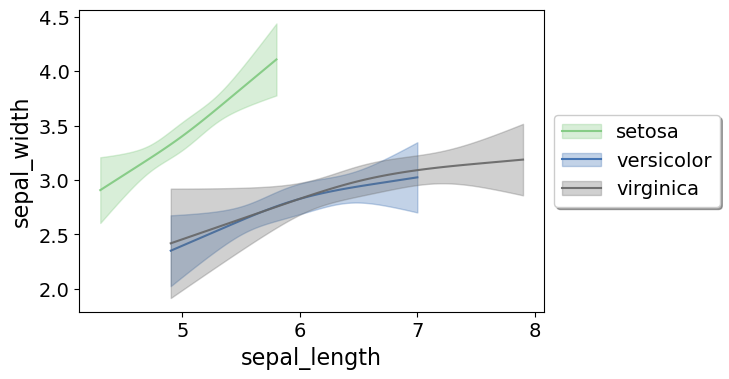If you pass in the `num_knots` argument, the knot locations are different for each subgroup of data (which I like as a default). If you pass in the `knots` argument and the locations, they are the same though for each subgroup.

Note that the way I estimate the models here I estimate three different models on the subsetted data frame, I do not estimate a stacked model with group interactions. So the error bands will be a bit wider than estimating the stacked model.

Sometimes superimposing many different groups is tough to visualize. So then a good option is to make a set of small multiple plots. To help with this, I’ve made a function `loc_error`, to pipe into seaborn’s small multiple set up:

``````#Small multiple example
g = sns.FacetGrid(iris, col='species',col_wrap=2)
g.map_dataframe(smooth.loc_error, x='sepal_length', y='sepal_width', num_knots=3)
g.set_axis_labels("Sepal Length", "Sepal Width")``````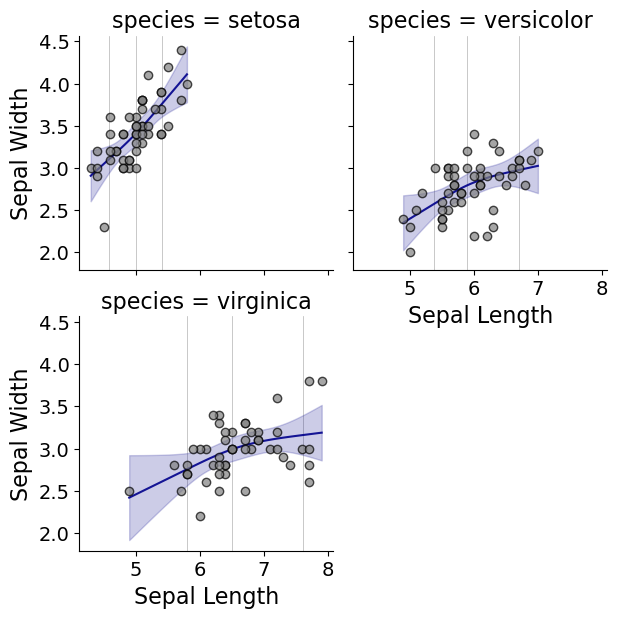And here you can see that the not locations are different for each subset, and this plot by default includes the original observations.

# Using the Formula Interface for Plots

Finally, I’ve been experimenting a bit with using the input in a formula interface, more similar to the way ggplot in R allows you to do this. So this is a new function, `plot_form`, and here is an example Poisson linear model:

``````smooth.plot_form(data=DC_crime,x='TotalLic',y='TotalCrime',
form='TotalCrime ~ TotalLic',
fam=sm.families.Poisson(), marker_size=12)``````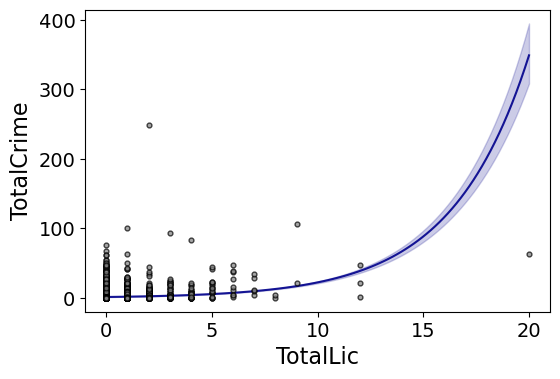You can see the explosive effect I talked about, which is common for Poisson/negative binomial models.

Here with the formula interface you can do other things, such as a polynomial regression:

``````#Can do polynomial terms
smooth.plot_form(data=DC_crime,x='TotalLic',y='TotalCrime',
form='TotalCrime ~ TotalLic + TotalLic**2 + TotalLic**3',
fam=sm.families.Poisson(), marker_size=12)``````

Which here ends up being almost indistinguishable from the linear terms. You can do other smoothers that are available in the patsy library as well, here are bsplines:

``````#Can do other smoothers
smooth.plot_form(data=DC_crime,x='TotalLic',y='TotalCrime',
form='TotalCrime ~ bs(TotalLic,df=4,degree=3)',
fam=sm.families.Poisson(), marker_size=12)``````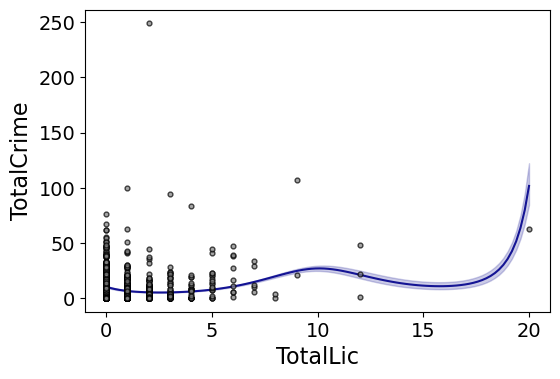I don’t really have a good reason to prefer restricted cubic splines to bsplines, I am just more familiar with restricted cubic splines (and this plot does not illustrate the knot locations that were by default chosen, although you could pass in knot locations to the `bs` function).

You can also do other transformations of the x variable. So here if you take the square root of the total number of licenses helps with the explosive effect somewhat:

``````#Can do transforms of the X variable
smooth.plot_form(data=DC_crime,x='TotalLic',y='TotalCrime',
form='TotalCrime ~ np.sqrt(TotalLic)',
fam=sm.families.Poisson(), marker_size=12)
``````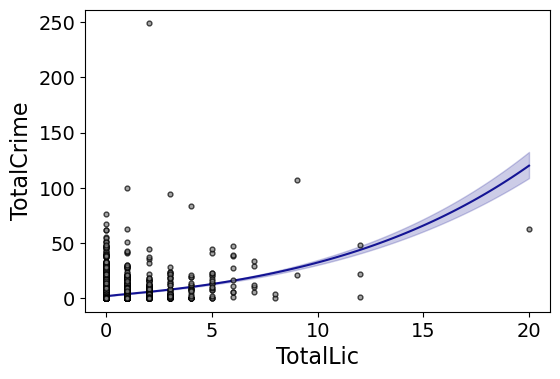In the prior blog post about explosive Poisson models I also showed a broken stick type model if you wanted to log the x variable but it has zero values.

``````#Can do multiple transforms of the X variable
smooth.plot_form(data=DC_crime,x='TotalLic',y='TotalCrime',
form='TotalCrime ~ np.log(TotalLic.clip(1)) + I(TotalLic==0)',
fam=sm.families.Poisson(), marker_size=12)``````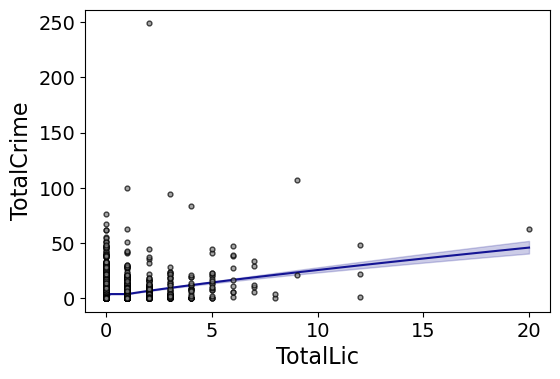Technically this “works” if you transform the Y variable as well, but the resulting plot is misleading, and the prediction interval is for the transformed variable. E.g. if you pass a formula `'np.log(TotalCrime+1) ~ TotalLic'`, you would need to exponentiate the the predictions and subtract 1 to get back to the original scale (and then the line won’t be the mean anymore, but the confidence intervals are OK).

I will need to see if I can figure out patsy and sympy to be able to do the inverse transformation to even do that. That type of transform to the y variable directly probably only makes sense for linear models, and then I would also maybe need to do a Duan type smearing estimate to get the mean effect right.

# Making aoristic density maps in R

I saw Jerry the other day made/updated an R package to do aoristic analysis. A nice part of this is that it returns the weights breakdown for individual cases, which you can then make maps of. My goto hot spot map for data visualization, kernel density maps, are a bit tough to work with weighted data though in R (tough is maybe not the right word, to use ggplot it takes a bit of work leveraging other packages). So here are some notes on that.

I have provided the data/code here. It is burglaries in Dallas, specifically I filter out just for business burglaries.

# R Code Snippet

First, for my front end I load the libraries I will be using, and change the working directory to where my data is located.

``````############################
library(aoristic) #aoristic analysis
library(rgdal)    #importing spatial data
library(spatstat) #weighted kde
library(raster)   #manipulate raster object
library(ggplot2)  #for contour graphs
library(sf)       #easier to plot sf objects

my_dir <- "D:\\Dropbox\\Dropbox\\Documents\\BLOG\\aoristic_maps_R\\data_analysis"
setwd(my_dir)
############################``````

Next I just have one user defined function, this takes an input polygon (the polygon that defines the borders of Dallas here), and returns a raster grid covering the bounding box. It also have an extra data field, to say whether the grid cell is inside/outside of the boundary. (This is mostly convenient when creating an RTM style dataset to make all the features conform to the same grid cells.)

``````###########################
#Data Manipulation Functions

#B is border, g is size of grid cell on one side
BaseRaster <- function(b,g){
base_raster <- raster(ext = extent(b), res=g)
projection(base_raster) <- crs(b)
mask_raster <- rasterize(b, base_raster, getCover=TRUE) #percentage of cover, 0 is outside
}
###########################``````

The next part I grab the datasets I will be using, a boundary file for Dallas (in which I chopped off the Lochs, so will not be doing an analysis of boat house burglaries today), and then the crime data. R I believe you always have to convert date-times when reading from a CSV (it never smartly infers that a column is date/time). And then I do some other data fiddling – Jerry has a nice function to check and make sure the date/times are all in order, and then I get rid of points outside of Dallas using the sp over function. Finally the dataset is for both residential/commercial, but I just look at the commercial burglaries here.

``````###########################
#Get the datasets

#Geo data
base_Dallas <- BaseRaster(b=boundary,g=200)
base_df <- as.data.frame(base_Dallas,long=TRUE,xy=TRUE)

#Crime Data
#prepping time fields
crime_dat\$Beg <- as.POSIXct(crime_dat\$StartingDateTime, format="%m/%d/%Y %H:%M:%OS")
crime_dat\$End <- as.POSIXct(crime_dat\$EndingDateTime, format="%m/%d/%Y %H:%M:%OS")

#cleaning up data
aor_check <- aoristic.datacheck(crime_dat, 'XCoordinate', 'YCoordinate', 'Beg', 'End')
coordinates(crime_dat) <- crime_dat[,c('XCoordinate', 'YCoordinate')]
crs(crime_dat) <- crs(boundary)
over_check <- over(crime_dat, boundary)
keep_rows <- (aor_check\$aoristic_datacheck == 0) & (!is.na(over_check\$city))
crime_dat_clean <- crime_dat[keep_rows,]

#only look at business burgs to make it go abit faster
busi_burgs <- crime_dat_clean[ crime_dat_clean\$UCROffense == 'BURGLARY-BUSINESS', ]
###########################``````

The next part preps the aoristic weights. First, the `aoristic.df` function is from Jerry’s aoristic package. It returns the weights broken down by 168 hours per day of the week. Here I then just collapse across the weekdays into the same hour, which to do that is simple, just add up the weights.

After that it is some more geographic data munging using the spatstat package to do the heavy lifting for the weighted kernel density estimate, and then stuffing the result back into another data frame. My bandwidth here, 3000 feet, is a bit large but makes nicer looking maps. If you do this smaller you will have a more bumpy and localized hot spots in the kernel density estimate.

``````###########################
#aoristic weights

#This takes like a minute
res_weights <- aoristic.df(busi_burgs@data, 'XCoordinate', 'YCoordinate', 'Beg', 'End')

#Binning into same hourly bins
for (i in 1:24){
cols <- (0:6*24)+i+5
lab <- paste0("Hour",i)
res_weights[,c(lab)] <- rowSums(res_weights[,cols])
}

#Prepping the spatstat junk I need
peval <- rasterToPoints(base_Dallas)[,1:2]
spWin <- as.owin(as.data.frame(peval))
sp_ppp <- as.ppp(res_weights[,c('x_lon','y_lat')],W=spWin) #spp point pattern object

#Creating a dataframe with all of the weighted KDE
Hour_Labs <- paste0("Hour",1:24)

for (h in Hour_Labs){
sp_den <- density.ppp(sp_ppp,weights=res_weights[,c(h)],
sigma=3000,
edge=FALSE,warnings=FALSE)
sp_dat <- as.data.frame(sp_den)
kd_raster <- rasterFromXYZ(sp_dat,res=res(base_Dallas),crs=crs(base_Dallas))
base_df[,c(h)] <- as.data.frame(kd_raster,long=TRUE)\$value
}
###########################``````

If you are following along, you may be wondering why all the hassle? It is partly because I want to use ggplot to make maps, but for its geom_contour it does not except weights, so I need to do the data manipulation myself to supply ggplot the weighted data in the proper format.

First I turn my Dallas boundary into a simple feature `sf` object, then I create my filled contour graph, supplying the regular grid X/Y and the Z values for the first Hour of the day (so between midnight and 1 am).

``````###########################
#now making contour graphs

dallas_sf <- st_as_sf(boundary)

#A plot for one hour of the day
hour1 <- ggplot() +
geom_contour_filled(data=base_df, aes(x, y, z = Hour1), bins=9) +
geom_sf(data=dallas_sf, fill=NA, color='black') +
scale_fill_brewer(palette="Greens") +
ggtitle('       Hour [0-1)') +
theme_void() + theme(legend.position = "none")
hour1

png('Hour1.png', height=5, width=5, units="in", res=1000, type="cairo")
hour1
dev.off()
###########################``````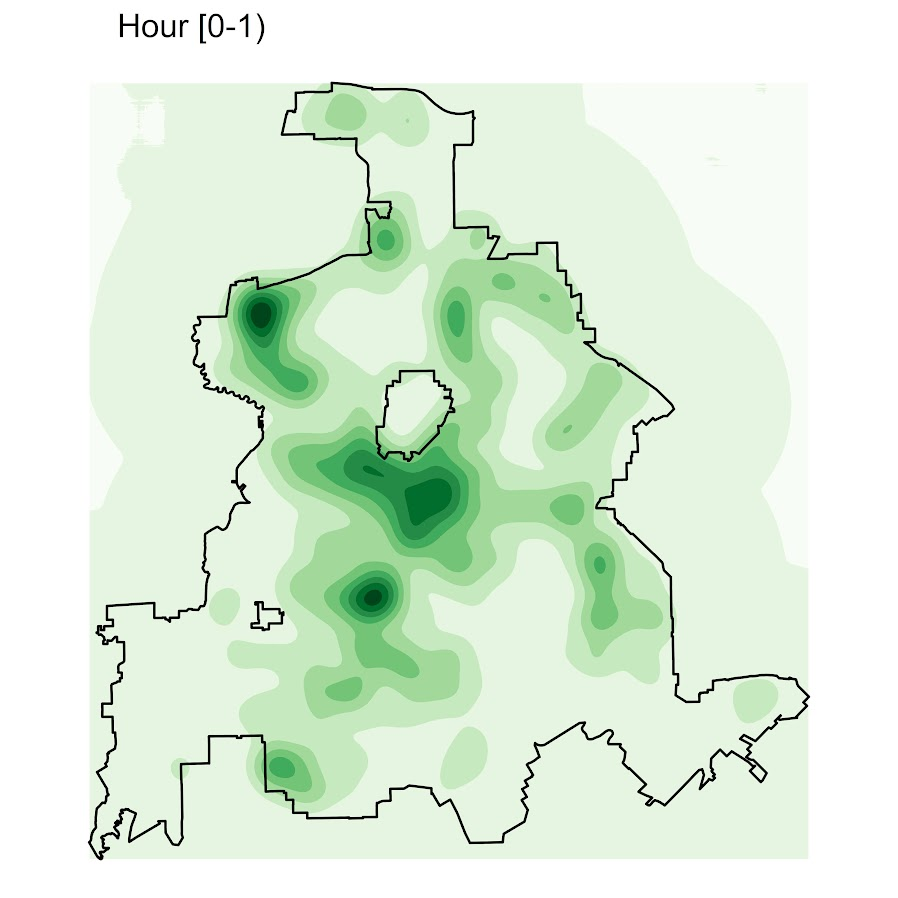Nice right! I have in the code my attempt to make a super snazzy small multiple plot, but that was not working out so well for me. But you can then go ahead and make up other slices if you want. Here is an example of taking an extended lunchtime time period.

``````###########################
#Plot for the afternoon time period
base_df\$Afternoon <- rowSums(base_df[,paste0("Hour",10:17)])

afternoon <- ggplot() +
geom_contour_filled(data=base_df, aes(x, y, z = Afternoon), bins=9) +
geom_sf(data=dallas_sf, fill=NA, color='black') +
scale_fill_brewer(palette="Greens") +
ggtitle('       Hour [9:00-17:00)') +
theme_void() + theme(legend.position = "none")
afternoon
###########################``````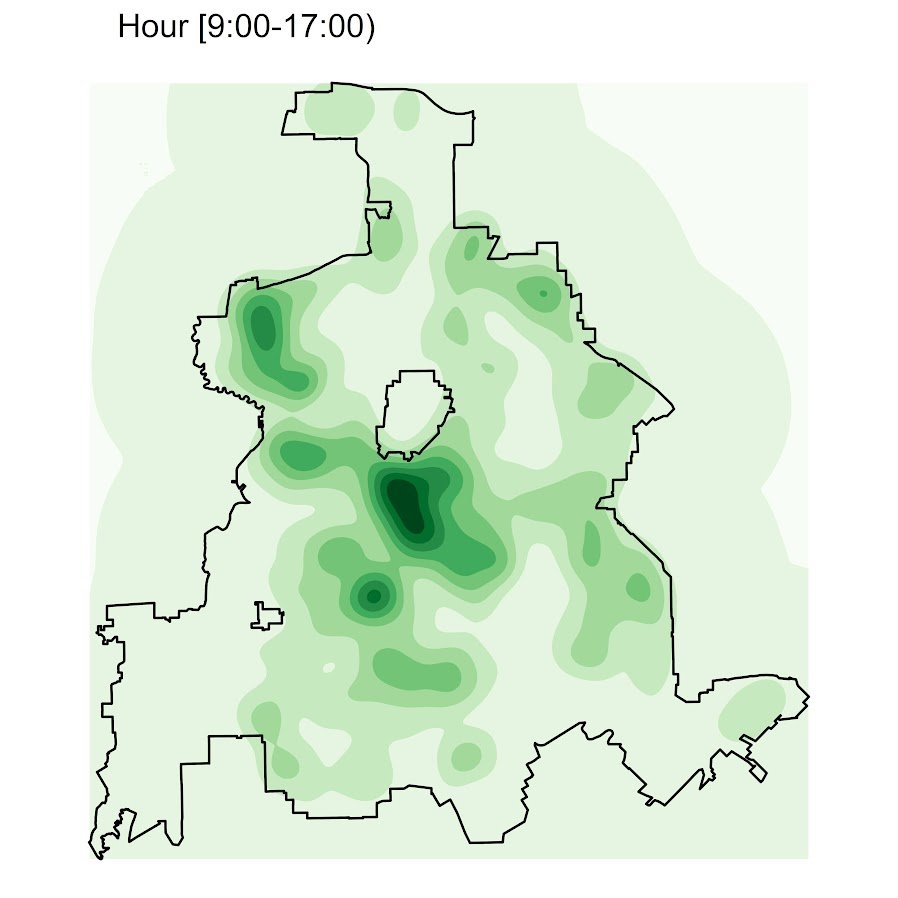So you can see that the patterns only slightly changed compared to the midnight prior graph.

Note that these plots will have different breaks, but you could set them to be equal by simply specifying a breaks argument in the `geom_contour_filled` call.

I will leave it up so someone who is more adept at R code than me to make a cool animated viz over time from this. But that is a way to mash up the temporal weights in a map.